• ## 深度神经网络

千次阅读 多人点赞 2018-11-28 15:15:23
深度神经网络 摘要：深度学习是近年来计算机人工智能领域非常火的研究方向，其相比传统的浅层机器学习而言能够挖掘出更多隐含的特征。深度学习现如今已经广泛的应用与计算机视觉、自然语言处理等领域，因此作为...
深度神经网络
摘要：深度学习是近年来计算机人工智能领域非常火的研究方向，其相比传统的浅层机器学习而言能够挖掘出更多隐含的特征。深度学习现如今已经广泛的应用与计算机视觉、自然语言处理等领域，因此作为计算机专业人工智能的学习者，学习和研究深度学习是一项必修课。
神经网络作为作为深度学习的“常客”，是成为构建深度学习框架的主要结构，神经网络以其与人类神经元相类似的元素通过相互连接形成网络拓扑结构，而这种模型能够自主挖掘更深层次特征。
建议深读的英文书籍：Neural Networks and Deep Learning

启发：线性回归
神经网络的结构
神经网络的训练
模型优化
其他经典的神经网络
TensorFlow实现标准神经网络源程序
总结

一、启发：线性回归
神经网络的基本计算主要以线性和非线性为主，线性是为了对不同的特征进行相互组合，其主要的运算结果是线性回归问题；非线性处理是为了对模型进行优化，使其能够处理非线性问题，其主要运算以函数形式。
线性模型是机器学习中最简单的分类模型，单变量线性回归是典型的回归问题，其主要模型为：
$\hat y = W^Tx+b$
其中 $W$ 表示参数矩阵（或权重矩阵），$b$ 表示偏向向量（一元线性曲线的截距），$x$ 表示输入的特征向量，$\hat y$ 表示对应的输出。线性分类器的目的便是训练一个线性回归方程，使得其能够拟合实际的样本输出。
通过曲线图可以描绘线性回归方程的含义：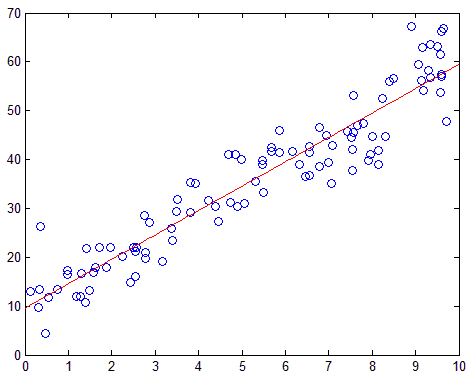下面给出模型训练的推导：
假设有一组训练样本，记作 $D=${($X$,$Y$)|$X=${$x_1,x_2,...,x_n$},$Y=${$y_1,y_2,...,y_n$}}，其中 $n$ 表示样本数，选择平方差作为损失函数，即：
$L(y,\hat y)=\frac{1}{2}|y-\hat y|^2$
则代价函数（所有样本的损失函数的均值）为：
$J(Y,\hat Y)=\frac{1}{2n}\sum|y-\hat y|^2$
构造了代价函数后，需要对其进行最优化处理，使得模型的代价尽可能降低。模型的优化方法常用的是梯度下降法（或称最速下降法）。梯度下降法通过对需要优化调整的参数进行调参。线性回归模型中需要调整的参数有权重矩阵$W$和偏向$b$，因此需要对这些参数求偏导，以获得梯度方向。
Ps：梯度下降法需要链式法则完成偏导的求解。
线性回归模型的梯度下降如下：
$\frac{\partial J}{\partial W^T}=\frac{\partial J}{\partial y^T}·\frac{\partial y^T}{\partial W^T}=\frac{1}{n}·(y-\hat y)·x$
$\frac{\partial J}{\partial b}=\frac{\partial J}{\partial y^T}·\frac{\partial y^T}{\partial b}=\frac{1}{n}·(y-\hat y)$
若选择学习率为 $\alpha(0<\alpha<1)$，则参数调整为：
$W^T:W^T-\alpha\frac{\partial J}{\partial W^T}$
$b:b-\alpha\frac{\partial J}{\partial b}$
通过不断循环调参，直到参数变化非常小的时候（在编程过程中，可以设置一个变量用以判断是否需要下一轮迭代），线性回归模型可以说训练完成。在评估该模型时候，仍然可以使用损失函数来对测试集进行评估，并计算相应的准确率、召回率、F1值等。
二、神经网络的结构
深入学习神经网络后会发，神经网络便是若干个线性回归方程的“相互交织”，再通过非线性方程进行“锐化”，因此第一节的线性回归问题是解决神经网络的基础。
神经网络是由神经元组成，神经元又称信息感知机，其通过多条路径（突触）接收外界信息并传导至神经细胞，并通过激活或抑制等策略做出反应。这里的突触即为数据的输入，神经细胞即为模型的结点，而反应即为激活函数。模型图如下所示：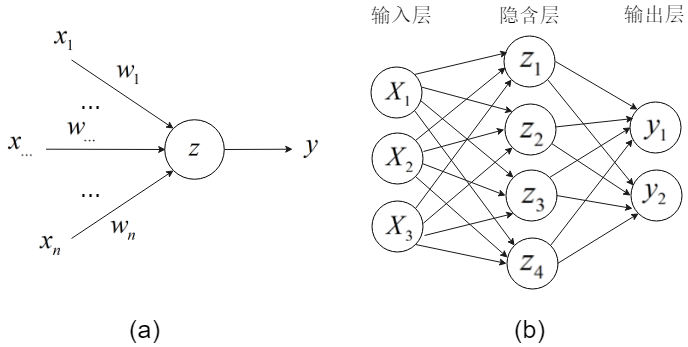图（a）为一个信息感知机，输入模型的数据为 $x_1,x_2,...,x_n$，连接的边上的权重分别为 $w_1,w_2,...,w_n$ ，结点神经细胞获取外界的输入记为：
$z=w_1x_1+w_2x_2+...+w_nx_n+b$
$z=W^T+b$
因此，对于单个神经元接受数据部分，是一个典型的线性回归模型。神经元的“激活或抑制反应”主要通过激活函数来完成。常用的激活函数有sigmod、tanh、ReLU、ELU等，这些激活函数都属于非线性函数，函数的输出即为该神经元对外做出的反应，即模型的输出值。以sigmod为例，该神经元的输出为：
$a=\sigma(W^T+b)=\frac{1}{1+e^{-(W^T+b)}}$
Ps：sigmod函数的性质：$\sigma'(z)=\sigma(z)(1-\sigma(z))$
图（b）是多个神经元相互交织在一起形成网络结构，其称为深度神经网络。深度神经网络常由输入层、隐含层和输出层组成。输入层的神经元个数等于输入样本的特征属性个数，隐含层可以自定个数（通常超过3个），隐含层的数据是不可见的，输出层作为模型的输出部分，神经元个数由需要分类的类标个数决定。
三、神经网络的训练
了解了神经网络的结构，需要了解神经网络如何进行训练。神经网络的训练过程分为如下几个步骤：

参数设置：设置模型的参数和超参数，包括权重矩阵、偏向、梯度下降的学习率；
前向传播：根据模型的输入层样本数据，计算每个神经元的接受数据和输出数据，并最终求得模型的输出层数据；
反向传播：选择损失函数并计算代价，选择优化策略（常选择梯度下降法）并进行调参；
模型的评估：根据测试集计算准确率、召回率、F1值等。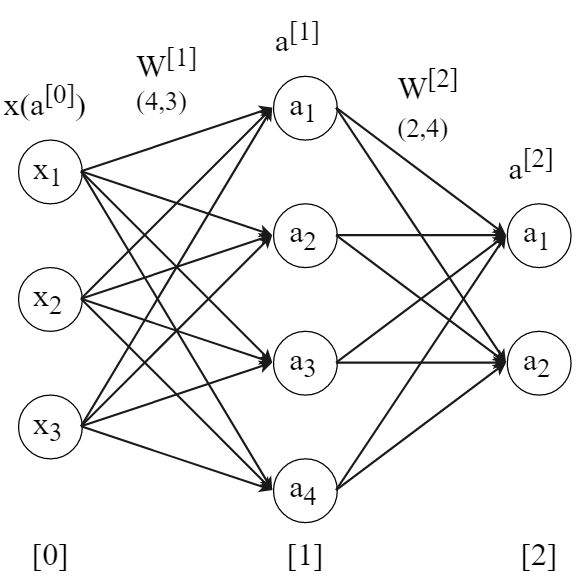假设一个深度神经网络的模型如上图所示，下面根据四步骤详解神经网络的训练过程。

1、参数设置
参数设置包括对需要学习的参数的初始化和对超参数（作为定值）的初始化。
（1）对需要学习的参数进行设置
假设神经网络输入层有三个神经元，隐含层有三个神经元，输出层有两个神经元，现在给定一组训练集，记作 $train=\{(X_1,Y_1),(X_2,Y_2),...,(X_N,Y_N)\}$，其中 $N$ 表示样本数量，$(X_i,Y_i)=(x{_1}{^{(i)}},x{_2}{^{(i)}},x{_3}{^{(i)}},y^{(i)})$，即每个样本有三个属性，分别对应神经网络的输入，每个样本对应一个真实类标（单类标问题），该类标有两个取值，设分别为0或1，即 $Y_i \in\mathbb \{0,1\}$，神经网络中的输出层有两个输出，分别对应两种取值的概率。
神经网络每条边都有一个权值，设输入层与隐含层之间的权值矩阵为
$W{^{}}= \begin{bmatrix} w{_{11}}{^{}} & w{_{21}}{^{}} & w{_{31}}{^{}} \\ w{_{12}}{^{}} & w{_{22}}{^{}} & w{_{32}}{^{}} \\ w{_{13}}{^{}} & w{_{23}}{^{}} & w{_{33}}{^{}} \\ w{_{14}}{^{}} & w{_{24}}{^{}} & w{_{34}}{^{}} \\ \end{bmatrix}$
$W{^{}}$ 矩阵的行数等于隐含层的神经元数，矩阵的列数等于输入层的神经元数，同理可得 $W{^{}}$：
$W{^{}}= \begin{bmatrix} w{_{11}}{^{}} & w{_{21}}{^{}} & w{_{31}}{^{}} & w{_{41}}{^{}} \\ w{_{12}}{^{}} & w{_{22}}{^{}} & w{_{32}}{^{}} & w{_{42}}{^{}} \\ \end{bmatrix}$
$W{^{}}$ 矩阵的行数等于输出层的神经元数，矩阵的列数等于隐含层的神经元数。
在进行计算或者编程过程中，弄清各个权重矩阵的维度对于后期的检查或调试至关重要。
隐含层和输出层偏向分别记为：
$b{^{}}=\begin{bmatrix}b{_1}{^{}} & b{_2}{^{}} & b{_3}{^{}} & b{_4}{^{}}\end{bmatrix}^T$
$b{^{}}=\begin{bmatrix}b{_1}{^{}} & b{_2}{^{}} & b{_3}{^{}} & b{_4}{^{}}\end{bmatrix}^T$
（2）对超参数进行设置
神经网络在训练过程中只有梯度下降法的学习率是不需要进行学习调整的参数，通常取0.05。
2、前向传播
神经网络的前向传播主要指输入层的数据顺着边流向隐含层，经过一次非线性处理后得到的数据再一次经过边流向输出层，经过处理后得到一组输出值。前向传播主要涉及到线性运算和非线性处理，在理解上非常简单。
（1）为了详细表达运算，先以单个样本进行前向传播的详细推导
根据第一节了解了线性回归的运算，如上图，隐含层的每一个神经元都将接收三条边传来的数据，并与该神经元本身的偏向相加，即有：
$z{{_1}{^{}}}=w{{_{11}}{^{}}}x_1+w{{_{21}}{^{}}}x_2+w{{_{31}}{^{}}}x_3+b{_1}{^{}}$
$z{{_2}{^{}}}=w{{_{12}}{^{}}}x_1+w{{_{22}}{^{}}}x_2+w{{_{32}}{^{}}}x_3+b{_2}{^{}}$
$z{{_3}{^{}}}=w{{_{13}}{^{}}}x_1+w{{_{23}}{^{}}}x_2+w{{_{33}}{^{}}}x_3+b{_3}{^{}}$
$z{{_4}{^{}}}=w{{_{14}}{^{}}}x_1+w{{_{24}}{^{}}}x_2+w{{_{34}}{^{}}}x_3+b{_4}{^{}}$
根据矩阵论的知识，可知这是一组多元线性方程组，可以用矩阵方式相乘，因此可以写作：
$z{^{}}=W{^{}}x+b{^{}}= \begin{bmatrix} w{_{11}}{^{}} & w{_{21}}{^{}} & w{_{31}}{^{}} \\ w{_{12}}{^{}} & w{_{22}}{^{}} & w{_{32}}{^{}} \\ w{_{13}}{^{}} & w{_{23}}{^{}} & w{_{33}}{^{}} \\ w{_{14}}{^{}} & w{_{24}}{^{}} & w{_{34}}{^{}} \\ \end{bmatrix} \begin{bmatrix} x{_1} \\ x{_2} \\ x{_3} \\ x{_4} \\ \end{bmatrix}+ \begin{bmatrix} b{_{1}}{^{}} \\ b{_{2}}{^{}} \\ b{_{3}}{^{}} \\ b{_{4}}{^{}} \\ \end{bmatrix}$
$z{^{}}$是一个4*1的向量，将其喂给sigmod函数后，得到隐含层的输出：
$a{^{}}=\sigma(z{^{}})$
其次，隐含层将数据传输给输出层，输入数据此时为 $a{^{}}$ ，与上面一样，可以得到：
$z{{_1}{^{}}}=w{{_{11}}{^{}}}a{_{1}}{^{}}+w{{_{21}}{^{}}}a{_{2}}{^{}}+w{{_{31}}{^{}}}a{_{3}}{^{}}+w{{_{41}}{^{}}}a{_{4}}{^{}}+b{_1}{^{}}$
$z{{_2}{^{}}}=w{{_{12}}{^{}}}a{_{1}}{^{}}+w{{_{22}}{^{}}}a{_{2}}{^{}}+w{{_{32}}{^{}}}a{_{3}}{^{}}+w{{_{42}}{^{}}}a{_{4}}{^{}}+b{_2}{^{}}$
即：
$z{^{}}=W{^{}}a{^{}}+b{^{}}= \begin{bmatrix} w{_{11}}{^{}} & w{_{21}}{^{}} & w{_{31}}{^{}} & w{_{41}}{^{}} \\ w{_{12}}{^{}} & w{_{22}}{^{}} & w{_{32}}{^{}} & w{_{42}}{^{}} \\ \end{bmatrix} \begin{bmatrix} a{_{1}}{^{}} \\ a{_{2}}{^{}} \\ a{_{3}}{^{}} \\ a{_{4}}{^{}} \\ \end{bmatrix}+ \begin{bmatrix} b{_{1}}{^{}} \\ b{_{2}}{^{}} \\ b{_{3}}{^{}} \\ b{_{4}}{^{}} \\ \end{bmatrix}$
最后，获得的输出喂给sigmoid函数（通常最后都喂给softmax函数，这里为了方便反向传播求导，仍然使用sigmoid函数），
$\hat y=a{^{}}=\sigma(z{^{}})$
因为该神经网络的输出层一共两个结点，因此对于单个样本，输出格式为[*，*]。
（2）对于多个样本，可以采用显示for循环方式按如上方式运算，但这样有明显两个缺点：一个是通过for循环显示进行循环非常耗时，第二是当数据量非常庞大时候对训练参数非常不利，因此对于多样本进行前向传播时，选择向量运算。假设样本集为 $X$，其含有$n$ 个样本。
（1）输入层样本：$X$，样本维度为（3，$n$）
（2）隐含层：$Z^{}=W^{}X+b^{}$，$A^{}=\sigma(Z{^{}})$，隐含层输出值维度为（4，$n$）；
（3）输出层：$Z^{}=W^{}A^{}+b^{}$，$A^{}=\sigma(Z{^{}})$，输出层输出值维度为（2，$n$）；
Ps：在编程时，样本集每一列表示一个样本。
3、反向传播
神经网络的训练关键就是训练它的权重矩阵和偏向，反向传播就是在不断地根据当前参数对样本产生的误差进行调整，以保证该误差尽可能小。反向传播时理解神经网络算法的核心。
神经网络反向传播常用的策略是梯度下降法，梯度下降法通过偏导数的链式法则实现反向传播，选择的损失函数是交差信息熵。为了详细推导梯度下降的反向传播，同样先以单个样本为例：
（1）单样本反向传播：根据单样本的前向传播推导，得到最终的输出值：
$\hat y=a^{}$
损失函数交差信息熵表达式是：
$L(y,\hat y)=L(y,a^{})=-[ylna^{}+(1-y)ln(1-a^{)})]$
因此可求出 $L$ 对 $a^{}$ 的偏导：
$\frac{\partial L}{\partial a^{}} = -\frac{y}{a^{}}+\frac{1-y}{1-a^{}}$
由 $\hat y=a{^{}}=\sigma(z{^{}})$ 式子可以继续求得 $L$ 对 $z^{}$ 的偏导，这里便需要链式求导：
$\frac{\partial L}{\partial z^{}} = \frac{\partial L}{\partial a^{}}\frac{\partial a^{}}{\partial z^{}} = (-\frac{y}{a^{}}+\frac{1-y}{1-a^{}})\sigma(z^{})(1-\sigma(z^{}))$
$=(-\frac{y}{a{^{}}}+\frac{1-y}{1-a{^{}}})a{^{}}(1-a{^{}})$
$=y(a{^{}}-1)+(1-y)a{^{}}=a{^{}}-y$
下面将要对权重矩阵和偏向进行求导，计算之前先进行必要的分析：隐层的神经元与输出层神经元相连的边都是唯一的，因此对于每一条边可通过链式法则求偏导：
以隐含层第一个神经元为例，先列出涉及到的前向传播的式子：
$z{{_1}{^{}}}=w{{_{11}}{^{}}}a{_{1}}{^{}}+...+b{_1}{^{}}$
$z{{_2}{^{}}}=w{{_{12}}{^{}}}a{_{1}}{^{}}+...+b{_2}{^{}}$
因此对于权重 $W{_{11}}{^{}}$ 偏导数为：
$\frac{\partial L}{\partial W{_{11}}{^{}}} = \frac{\partial L}{\partial z{_{1}}^{}}\frac{\partial z{_{1}}^{}}{\partial W{_{11}}{^{}}} = (a{_{1}}{^{}}-y)a{_{1}}{^{T}}$
对于权重 $W{_{12}}{^{}}$ 偏导数为：
$\frac{\partial L}{\partial W{_{12}}{^{}}} = \frac{\partial L}{\partial z{_{2}}^{}}\frac{\partial z{_{2}}^{}}{\partial W{_{12}}{^{}}} = (a{_{2}}{^{}}-y)a{_{1}}{^{T}}$
因此可以归纳出一个对于边 $W{_{ij}}{^{}}$ 的偏导通向公式：
$\frac{\partial L}{\partial W{_{ij}}{^{}}} = \frac{\partial L}{\partial z{_{j}}^{}}\frac{\partial z{_{j}}^{}}{\partial W{_{ij}}{^{}}} = (a{_{j}}{^{}}-y)a{_{i}}{^{T}}$
对于输出层的偏向，以输出层第一个神经元为例，列出涉及到的前向传播一个式子：
$z{{_1}{^{}}}=w{{_{11}}{^{}}}a{_{1}}{^{}}+w{{_{21}}{^{}}}a{_{2}}{^{}}+w{{_{31}}{^{}}}a{_{3}}{^{}}+w{{_{41}}{^{}}}a{_{4}}{^{}}+b{_1}{^{}}$
，由前向传播式子可以很简单的求出导数为：
$\frac{\partial L}{\partial b{_{1}}{^{}}} = \frac{\partial L}{\partial z{_{1}}^{}}\frac{\partial z{_{1}}^{}}{\partial b{_{1}}{^{}}} = a{_{1}}{^{}}-y$
$\frac{\partial L}{\partial b{_{2}}{^{}}} = \frac{\partial L}{\partial z{_{2}}^{}}\frac{\partial z{_{2}}^{}}{\partial b{_{2}}{^{}}} = a{_{2}}{^{}}-y$
对于隐含层的求导较为复杂，如下图，对于隐含层的某一个神经元，其将收到所有输出层神经元的反向传播，因此对于隐含层的每一个神经元的输出值求导需要分别对所有输出层进行偏导求和。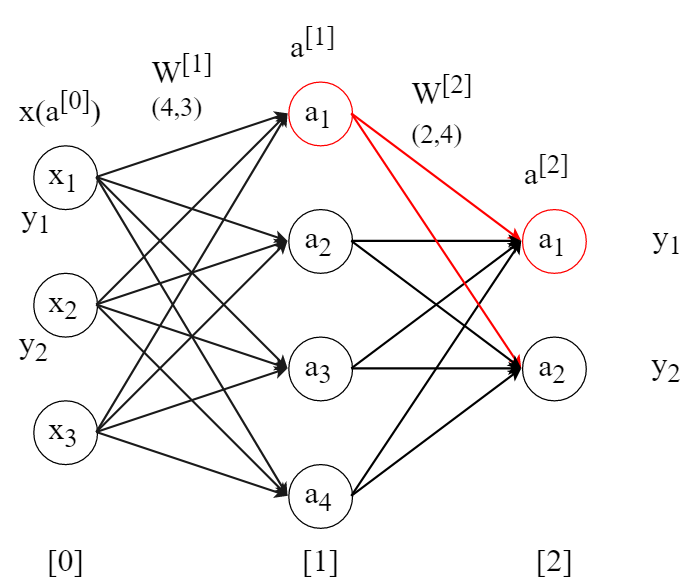涉及到的前向传播式子还是这个：
$z{{_1}{^{}}}=w{{_{11}}{^{}}}a{_{1}}{^{}}+...+b{_1}{^{}}$
$z{{_2}{^{}}}=w{{_{12}}{^{}}}a{_{1}}{^{}}+...+b{_2}{^{}}$
因此可求出 $L$ 对 $a{_1}{^{}}$ 的偏导：
$\frac{\partial L}{\partial a{_1}{^{}}} = \frac{\partial L}{\partial z{_1}{^{}}}\frac{\partial z{_1}{^{}}}{\partial a{_1}{^{}}} +\frac{\partial L}{\partial z{_2}{^{}}}\frac{\partial z{_2}{^{}}}{\partial a{_1}{^{}}}$
$=(a{_{1}}{^{}}-y)w{{_{11}}{^{}}}+(a{_{2}}{^{}}-y)w{{_{12}}{^{}}}$
由此可以列出另三个：
$\frac{\partial L}{\partial a{_2}{^{}}} = \frac{\partial L}{\partial z{_1}{^{}}}\frac{\partial z{_1}{^{}}}{\partial a{_2}{^{}}} +\frac{\partial L}{\partial z{_2}{^{}}}\frac{\partial z{_2}{^{}}}{\partial a{_2}{^{}}}$
$=(a{_{1}}{^{}}-y)w{{_{21}}{^{}}}+(a{_{2}}{^{}}-y)w{{_{22}}{^{}}}$
$\frac{\partial L}{\partial a{_3}{^{}}} = \frac{\partial L}{\partial z{_1}{^{}}}\frac{\partial z{_1}{^{}}}{\partial a{_3}{^{}}} +\frac{\partial L}{\partial z{_2}{^{}}}\frac{\partial z{_2}{^{}}}{\partial a{_3}{^{}}}$
$=(a{_{1}}{^{}}-y)w{{_{31}}{^{}}}+(a{_{2}}{^{}}-y)w{{_{32}}{^{}}}$
$\frac{\partial L}{\partial a{_4}{^{}}} = \frac{\partial L}{\partial z{_1}{^{}}}\frac{\partial z{_1}{^{}}}{\partial a{_4}{^{}}} +\frac{\partial L}{\partial z{_2}{^{}}}\frac{\partial z{_2}{^{}}}{\partial a{_4}{^{}}}$
$=(a{_{1}}{^{}}-y)w{{_{41}}{^{}}}+(a{_{2}}{^{}}-y)w{{_{42}}{^{}}}$
合并起来可以以矩阵形式呈现：
$\frac{\partial L}{\partial a{^{}}} = \frac{\partial L}{\partial z{_1}{^{}}}\frac{\partial z{_1}{^{}}}{\partial a{^{}}} +\frac{\partial L}{\partial z{_2}{^{}}}\frac{\partial z{_2}{^{}}}{\partial a{^{}}}= \begin{bmatrix} w{_{11}}{^{}} & w{_{12}}{^{}} \\ w{_{21}}{^{}} & w{_{22}}{^{}} \\ w{_{31}}{^{}} & w{_{32}}{^{}} \\ w{_{41}}{^{}} & w{_{42}}{^{}} \\ \end{bmatrix} \begin{bmatrix} a{_{1}}{^{}}-y \\ a{_{2}}{^{}}-y \\ \end{bmatrix} =w^{T}a^{}$
有了 $L$ 对 $a{^{}}$ 的偏导，则可以求出 $L$ 对 $z{^{}}$ 的偏导：
$\frac{\partial L}{\partial z{^{}}}=\frac{\partial L}{\partial a{^{}}} \frac{\partial a{^{}}}{\partial z{^{}}} = w^{T}a^{}a^{}(1-a^{})$
对于输入层与隐含层之间的权重矩阵和隐含层的偏向的求导，方法与上面的一致：
对于边 $W{_{ij}}{^{}}$ 的偏导通向公式：
$\frac{\partial L}{\partial W{_{ij}}{^{}}} = \frac{\partial L}{\partial z{_{j}}^{}}\frac{\partial z{_{j}}^{}}{\partial W{_{ij}}{^{}}} = w{_j}{^{T}}a{_j}{^{}}a{_j}{^{}}(1-a{_j}{^{}})a{_{i}}{^{T}}$
对于隐含层偏向 $b{_{j}}{^{}}$ 的偏导通向公式：
$\frac{\partial L}{\partial b{_{j}}{^{}}} = \frac{\partial L}{\partial z{_{1}}^{}}\frac{\partial z{_{1}}^{}}{\partial b{_{j}}{^{}}} = w{_j}{^{T}}a{_j}{^{}}a{_j}{^{}}(1-a{_j}{^{}})$
到此，关于神经网络的权重矩阵和偏向的梯度求解全部结束，参数的调整即为：
$w:w-\alpha\frac{\partial J}{\partial w}$
$b:b-\alpha\frac{\partial J}{\partial b}$
四、模型优化
神经网络在实际使用中，其深度不只有三层，而是大于三层，输入层。隐含层和输出层的结点也都有几十上百个神经元，因此对于一个神经网络来说，模型的参数是庞大的，因此传统的模型在一般的机器上很难快速训练参数，因此在深度神经网络的训练过程中，如何对模型进行优化成为比较现实的问题。
模型优化有几个方面：防止模型过拟合、选择其他的激活函数、选择其他的损失函数或不同的优化模型等。
1、正则化
正则化是最常用的防止过拟合的策略，其主要在代价函数后添加正则参数，如下：
$J(w,b)=\frac{1}{m} \sum L(y,\hat y)+\frac{\lambda}{2m}\sum||w||{^{2}}$
其中 $\frac{\lambda}{2m}\sum||w||{^{2}}$ 为L2正则化，$\lambda$ 称为正则化参数。现对代价函数进行求导，可得：
$\frac{\partial J}{\partial w}=\frac{1}{m} \frac{\partial L}{\partial w}+\frac{\lambda}{m} w$
因此权重矩阵的参数调整为：
$w:w-\alpha\frac{\partial J}{\partial w}=w-\alpha(\frac{1}{m} \frac{\partial L}{\partial w}+\frac{\lambda}{m}w)$
$=w-\frac{\alpha}{m}\frac{\partial L}{\partial w}-\frac{\alpha \lambda}{m}w=(1-\frac{\alpha \lambda}{m})w-\frac{\alpha}{m}\frac{\partial L}{\partial w}$
为什么正则化可以防止过拟合？以 $tanh(z)$ 激活函数为例，tanh激活函数的函数图像为：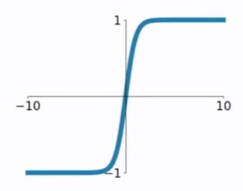可以发现，当参数 $z$ 很小的时候，函数近乎为线性，而对代价函数调价正则化后，若 $\lambda$的值越大，则权重矩阵 $w$ 的各个元素的值越小（甚至为0），因此由 $z=wx+b$ 可知 $z$ 的值变小。所以在带入tanh激活函数中时，其近乎为线性函数，因此实现了防止过拟合。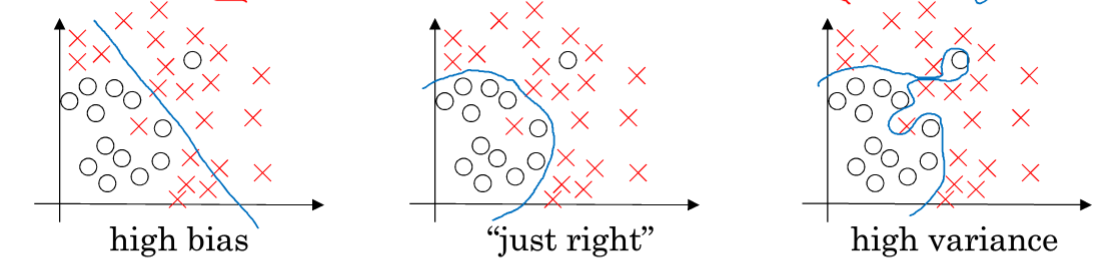如上图，左图属于欠拟合（高偏差），右图属于过拟合（高方差），正则化后属于中间的图。
其他正则化还有dropout，可以实现防止过拟合。
2、激活函数
激活函数包括sigmod、tanh、ReLU、ELU等，激活函数的选择在一定程度上会影响模型的训练效果。关于激活函数的详解，可参考其他内容。
3、梯度问题
由于神经网络的数据量庞大问题，除了产生过拟合，还会产生梯度衰减或梯度爆炸问题，由于梯度下降法的链式求导原则，可能会造成参数调整后过大，或趋近于0。解决梯度衰减和梯度爆炸问题，对于不同类型的网络有不同的解决方案，常用的解决方案是更换激活函数。
五、其他经典的神经网络
深度神经网络，又称为BP神经网络或者前馈神经网络，其属于标准的神经网络模型，除了这个模型外，还有其他许多类型神经网络。
1、循环神经网络RNN
循环神经网络通常可以解决序列问题。传统的BP神经网络的输入层神经元个数取决于输入样本的特征属性个数，且输入样本之间没有联系。而对于自然语言处理中相关的问题（情感分析、词性标注、命名实体识别、语音识别等）的输入数据之间往往是有关联的。例如在命名实体识别的任务中，要识别一句话“马云在浙江杭州创办了阿里巴巴”，如果单纯的对于每一个字作为神经网络的输入，模型是无法进行准确的识别，因为对于实体来说只有多个字组合起来才能形成实体，因此RNN用来解决序列问题。
关于RNN的变种还有双向循环神经网络、长短期记忆神经网络（LSTM）、双向长短期记忆神经网络（Bi-LSTM）等。
2、卷积神经网络CNN
卷积神经网络常用来进行图像处理，其通过将图像的数据（（m，n，3），其中m，n为图像像素，3为三原色）进行卷积操作，再通过池化层（最大池化或平均池化）进行降维，最后通过softmax层取出最大值作为特征。卷积神经网络也可以用在文本处理中。
六、TensorFlow实现标准神经网络源程序
为了深入学习神经网络的实现过程，本人使用python的TensorFlow框架实现了BP神经网络算法，编程采用python面向对象，程序的运行流程如图：
数据处理数据处理读取数据集训练集测试集模型训练预测
1. main函数：实现读取训练集和测试集文件，并对数据进行处理（分为训练集X和y、测试集X和y），并调用神经网络类进行训练和预测。
#code by WangJianing
#time:2018.11.24

import tensorflow as tf
import numpy as np
from neural_network import NN

"""
"""
input_x = []
input_y = []
with open(filename,'r') as f:
while True:
if line == '':
break
else:
line = line.replace('\n','')
sample = line.split(' ')
x = sample[0:3]
x = list(map(np.float32, x))
y = sample
y = list(map(np.int32, y))
input_x.append(x)
input_y.append(y)
return input_x,input_y

if __name__ == '__main__':
config = tf.ConfigProto()
config.gpu_options.allow_growth = True
config.gpu_options.per_process_gpu_memory_fraction = 0.2  # need ~700MB GPU memory
sample_size = [len(train_y),len(test_y)]
print(sample_size)
train_x = np.transpose(train_x)
input_y = np.zeros([2,sample_size])
test_x = np.transpose(test_x)
test_y = np.transpose(test_y)
for ei,i in enumerate(train_y):
input_y[i][ei]=1
# print(ei,i)
#build neural network
n = NN(train_x, input_y, test_x, test_y, 'GradientDescentOptimizer', sample_size, config, learning_rate=0.05)
#train
n.train1()
#test
n.test()

2. 神经网络类：tensorflow创建计算图结点，神经网络参数初始化、前向传播、反向传播、最小化损失函数和梯度下降调参、测试集预测和精度评估。
#code by WangJianing
#time:2018.11.24

import tensorflow as tf
import numpy as np

class NN(object):
"""docstring for NN"""
def __init__(self, train_x, train_y, test_x, test_y, optimize, sample_size, config, learning_rate=0.05):
super(NN, self).__init__()
self.train_x = tf.to_float(train_x, name='ToFloat1')
self.train_y = tf.to_float(train_y, name='ToFloat2')
self.test_x = tf.to_float(test_x, name='ToFloat3')
self.test_y = tf.to_float(test_y, name='ToFloat4')
self.learning_rate = learning_rate
self.optimize = optimize
self.sess = tf.Session()
self.sample_size = sample_size
self.config = config
self.para = [[],[],[],[],0]
self.bildGraph()
# self.train()

def bildGraph(self):
self.parameter_op()
self.towards_op()
self.loss_op()
self.backwords_op()
# self.test_towords()
self.init_op()

def testBuildGraph(self):
self.parameter_op()
self.towards_op()

def parameter_op(self):
self.weight1 = tf.Variable(tf.random_normal([4, 3], stddev=0.03), dtype=tf.float32, name='weight1')
self.bias1 = tf.Variable(tf.random_normal([4, 1]), dtype=tf.float32, name='bias1')
self.weight2 = tf.Variable(tf.random_normal([2, 4], stddev=0.03), dtype=tf.float32, name='weight2')
self.bias2 = tf.Variable(tf.random_normal([2, 1]), dtype=tf.float32, name='bias2')
self.input_xx = tf.Variable(self.train_x,name='xx1')
self.input_xx_test = tf.Variable(self.test_x,name='xx3')
self.input_yy = tf.Variable(self.train_y,name='xx2')

def appendVector(self, v, size, kind):
#该方法是将一个一维向量v复制size次并拼起来
_v = tf.transpose(v)
# print('_v=',_v)
new_v = []
if kind == 0:
for i in range(size):
new_v.append(_v)
self.bias1_train = tf.Variable(new_v, dtype=tf.float32, name='bias1_train')
self.bias1_train = tf.transpose(self.bias1_train)
elif kind == 1:
for i in range(size):
new_v.append(_v)
self.bias2_train = tf.Variable(new_v, dtype=tf.float32, name='bias2_train')
self.bias2_train = tf.transpose(self.bias2_train)
elif kind == 2:
for i in range(size):
new_v.append(_v)
self.bias1_test = tf.Variable(new_v, dtype=tf.float32, name='bias1_test')
self.bias1_test = tf.transpose(self.bias1_test)
elif kind == 3:
for i in range(size):
new_v.append(_v)
self.bias2_test = tf.Variable(new_v, dtype=tf.float32, name='bias2_test')
self.bias2_test = tf.transpose(self.bias2_test)

def towards_op(self):
self.m1 = tf.matmul(self.weight1, self.input_xx, name='matmul1')
# print('m1=',self.m1)
self.appendVector(self.bias1, self.sample_size, 0)
# print('self.bias1_train=',self.bias1_train)
self.a1 = tf.nn.sigmoid(self.z1,name='a1')
self.appendVector(self.bias2, self.sample_size, 1)
self.z2 = tf.add(tf.matmul(self.weight2, self.a1, name='matmul2'),self.bias2_train, name='z2')
self.a2 = tf.transpose(tf.nn.softmax(tf.transpose(self.z2,[1,0]),name='a2'),[1,0])

def test_towords(self):
self.t_m1 = tf.matmul(self.para, self.input_xx_test, name='matmul3')
self.appendVector(self.para, self.sample_size, 2)
self.t_a1 = tf.nn.sigmoid(self.t_z1,name='a1')
self.appendVector(self.para, self.sample_size, 3)
self.t_z2 = tf.add(tf.matmul(self.para, self.t_a1, name='matmul4'),self.bias2_test, name='z2')
self.t_a2 = tf.transpose(tf.nn.softmax(tf.transpose(self.t_z2,[1,0]),name='a2'),[1,0])

def loss_op(self):
self.loss = tf.reduce_mean(tf.nn.sigmoid_cross_entropy_with_logits(labels=self.train_y, logits=self.a2))

def backwords_op(self):
self.train = self.optimizer.minimize(self.loss)

def train1(self):
with tf.Session(config=tf.ConfigProto(gpu_options=tf.GPUOptions(per_process_gpu_memory_fraction=0.333))) as sess:
sess.run(self.init_op)
for i in range(10):
sess.run(self.train)
self.para = [sess.run(self.weight1),sess.run(self.weight2),sess.run(self.bias1),sess.run(self.bias2),sess.run(self.loss)]
print("==========step",i,"==========")
print("weight1:\n",self.para,"\nb1:\n",self.para)
print("\nweight2:\n",self.para,"\nb2:\n",self.para)
print("\nloss=",self.para)

def init_op(self):
self.init_op = tf.global_variables_initializer()

def test(self):
self.test_towords()
with tf.Session(config=tf.ConfigProto(gpu_options=tf.GPUOptions(per_process_gpu_memory_fraction=0.333))) as sess:
sess.run(tf.global_variables_initializer())
sess.run([self.bias1_test,self.bias2_test])
#每个样本的每个类标取值的概率
predict_proba = sess.run(self.t_a2)
#预测每个样本的类标（0或1）
predict_proba = np.transpose(predict_proba)
print('\npredict_proba=',predict_proba)
predict_value = np.argmax(predict_proba,axis=1)
print('\npredic_value=',predict_value)
#计算准确率：
# accuracy = 0
# # print(test_y)
# for ei,i in enumerate(predict_value):
#     if i == self.test_y[ei]:
#         accuracy += 1
# accuracy  /= sample_size
# print('\naccuracy=',accuracy)

七、总结
本人在编写本博文大概花了一周时间，经过对深度学习的常用模型——神经网络的原理理解、模型训练的推导以及最后程序编写，能够更加深刻的理解神经网络模型，这对今后学习机器学习、深度学习以及涉及到相关应用时具有很大的作用。
深度学习的不断发展，将越来越为生产生活提供便利，学好神经网络，是走入深度学习的必经之路，能够带着理解走入人工智能领域，对工程、科研具有巨大的意义。
本人将继续在人工智能、机器学习、深度学习领域内不断学习，不断更新博文。
博客记录着学习的脚步，分享着最新的技术，非常感谢您的阅读，本博客将不断进行更新，希望能够给您在技术上带来帮助。


展开全文深度学习 神经网络 机器学习
• ## 深度神经网络总结

万次阅读 多人点赞 2019-01-09 17:52:24
深度神经网络（Deep Neural Networks，DNN）可以理解为有很多隐藏层的神经网络，又被称为深度前馈网络（DFN），多层感知机（Multi-Layer perceptron,MLP）。 1 前向传播算法 1.1 从感知机到神经网络 感知机的模型...
深度神经网络（Deep Neural Networks，DNN）可以理解为有很多隐藏层的神经网络，又被称为深度前馈网络（DFN），多层感知机（Multi-Layer perceptron,MLP）。

1 前向传播算法

1.1 从感知机到神经网络

感知机的模型是一个有若干输入和一个输出的模型，如下图: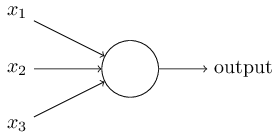输出和输入之间学习到一个线性关系，得到中间输出结果：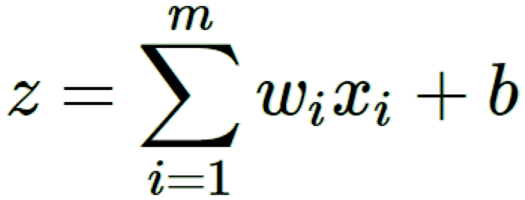接着是一个神经元激活函数，得到输出结果1或者-1。: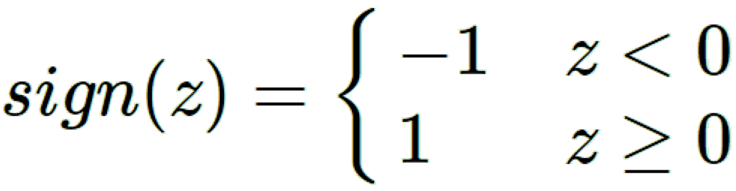这个模型只能用于二元分类，且无法学习比较复杂的非线性模型，因此在工业界无法使用。

而神经网络则在感知机的模型上做了扩展，总结下主要有三点：

1）加入了多层隐藏层，增强模型的表达能力。
2）输出层神经元可以不止一个，可以有多个输出，这样模型可以灵活的应用于分类,回归，降维和聚类等。下图输出层有4个神经元。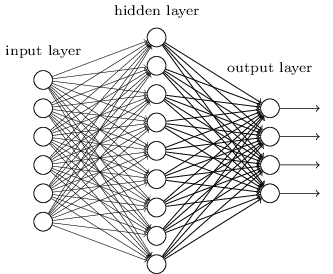3） 对激活函数做扩展。感知机的激活函数是sign(z),虽然简单但是处理能力有限，因此神经网络中一般使用：Sigmoid，tanx, ReLU，softplus，softmax等加入非线性因素，提高模型的表达能力。
1.2 DNN的基本结构

按不同层的位置划分，DNN内部的神经网络层可以分为：输入层，隐藏层和输出层，一般第一层是输入层，最后一层是输出层，而中间的层数都是隐藏层。层与层之间是全连接的，即第i层的任意一个神经元一定与第i+1层的任意一个神经元相连。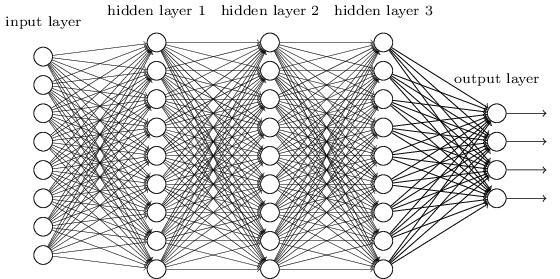虽然DNN看起来很复杂，但是从小的局部模型来说，还是和感知机一样，即一个线性关系加上一个激活函数σ(z)。

由于DNN层数多，参数较多，线性关系系数w和偏倚b的定义需要一定的规则。线性关系系数w的定义：第二层的第4个神经元到第三层的第2个神经元的线性系数定义为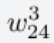。上标3代表线性系数w所在的层数，而下标对应的是输出的第三层索引2和输入的第二层索引4。你也许会问，为什么不是w342, 呢？这主要是为了便于模型用于矩阵表示运算，如果是w342而每次进行矩阵运算是wTx+b，需要进行转置。将输出的索引放在前面的话，则线性运算不用转置,即直接为wx+b。注意，输入层是没有w参数，偏倚参数b。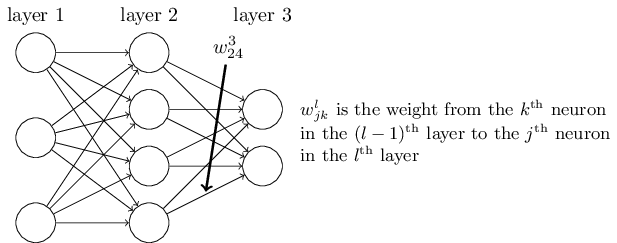偏倚b的定义：第二层的第三个神经元对应的偏倚定义为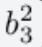。其中，上标2代表所在的层数，下标3代表偏倚所在的神经元的索引。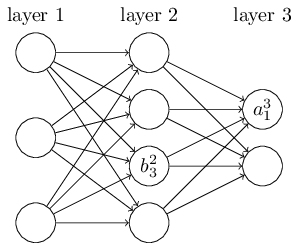1.3 DNN前向传播算法数学原理

假设选择的激活函数是σ(z)，隐藏层和输出层的输出值为a。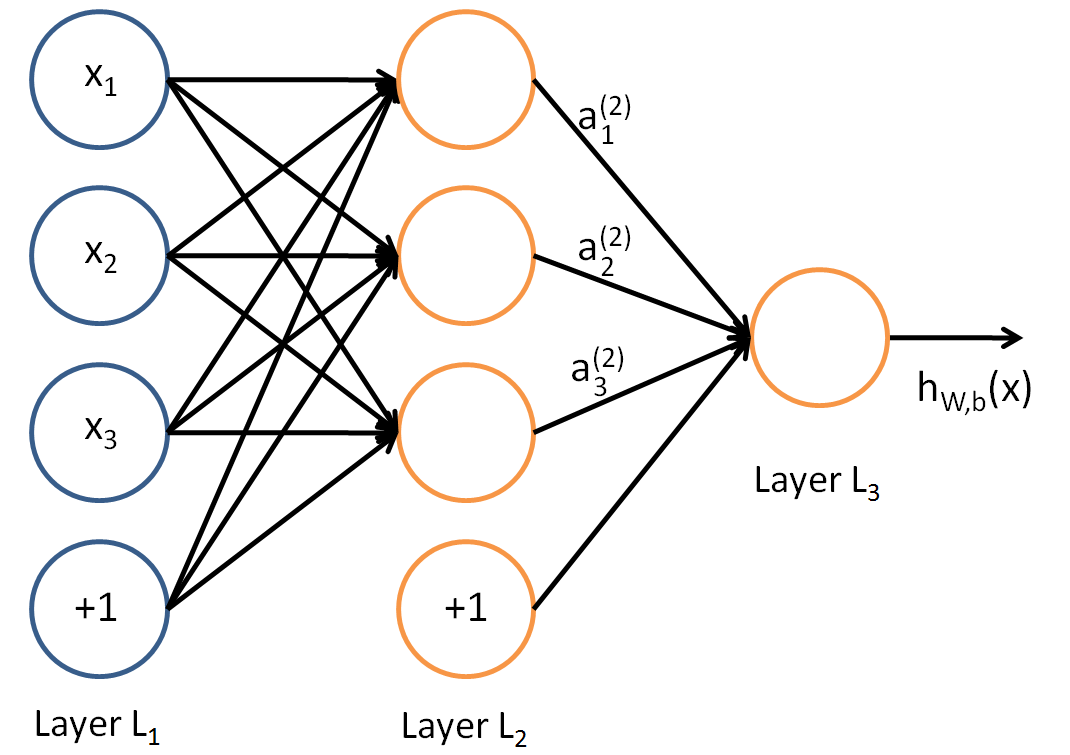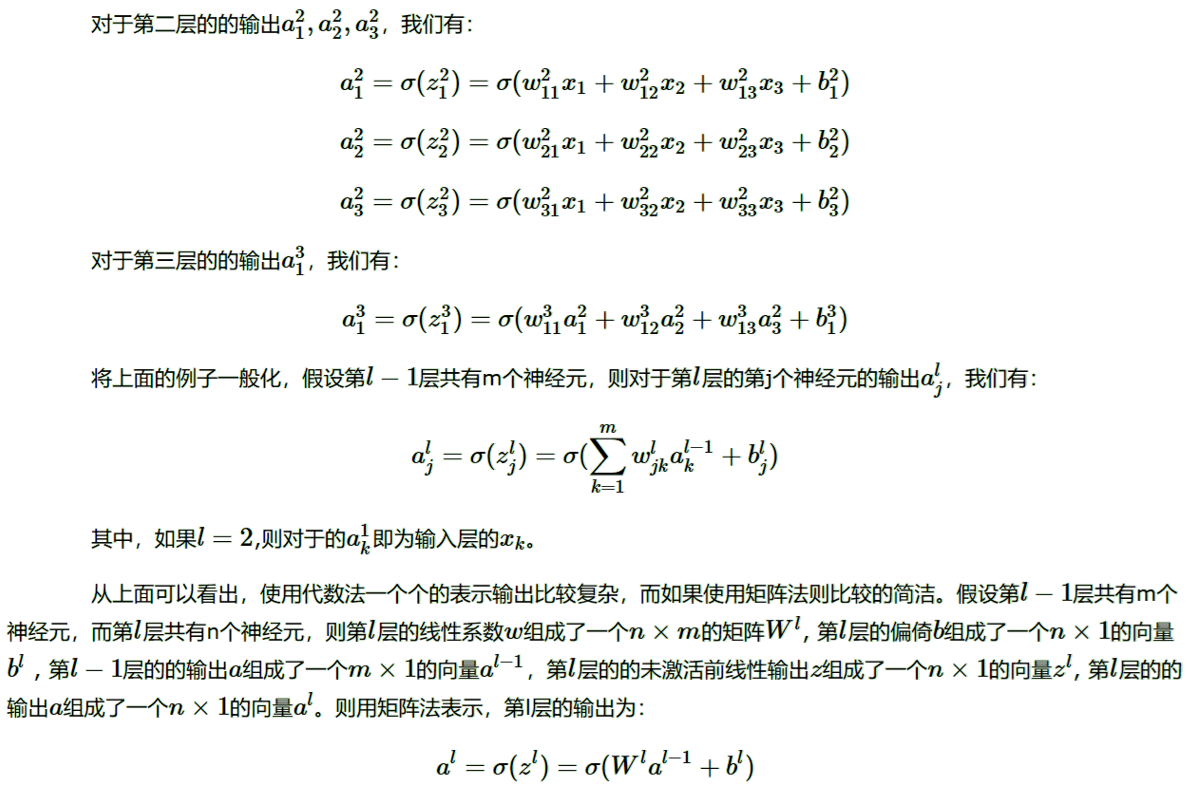1.4 DNN前向传播算法

DNN的前向传播算法是利用若干个权重系数矩阵W,偏倚向量b来和输入值向量x进行一系列线性运算和激活运算，从输入层开始，利用上一层的输出计算下一层的输出，一层层的向后计算，一直到运算到输出层，得到输出结果为值。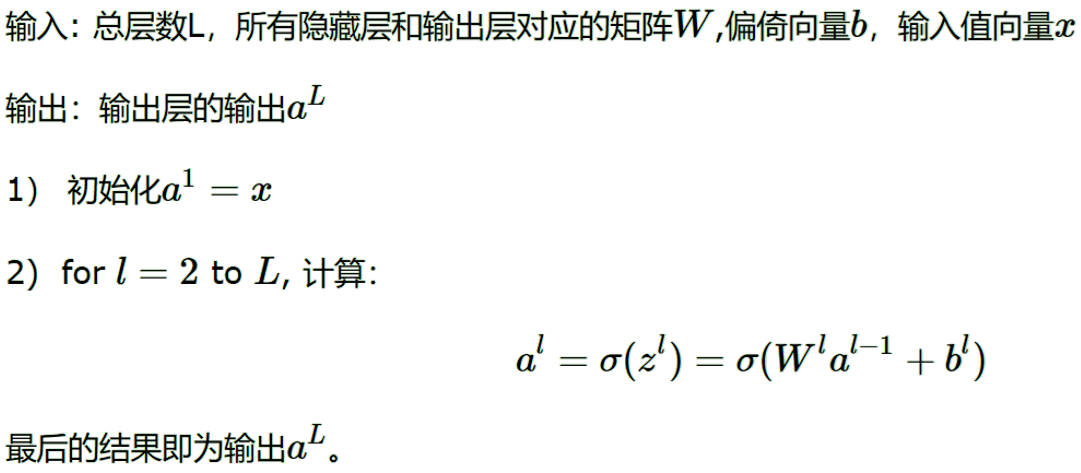2 DNN反向传播算法

使用前向传播计算训练样本的输出，使用损失函数，来度量训练样本计算出的输出和真实的训练样本标签之间的损失。DNN的反向传播算法（Back Propagation，BP）通过对损失函数用梯度下降法进行迭代优化求极小值，找到合适的隐藏层和输出层对应的线性系数矩阵W,偏倚向量b,让所有的训练样本输入计算出的输出尽可能的等于或接近样本标签。

2.1 DNN反向传播算法的基本思路

使用均方差来度量损失进行推导。即对于每个样本，期望最小化下式：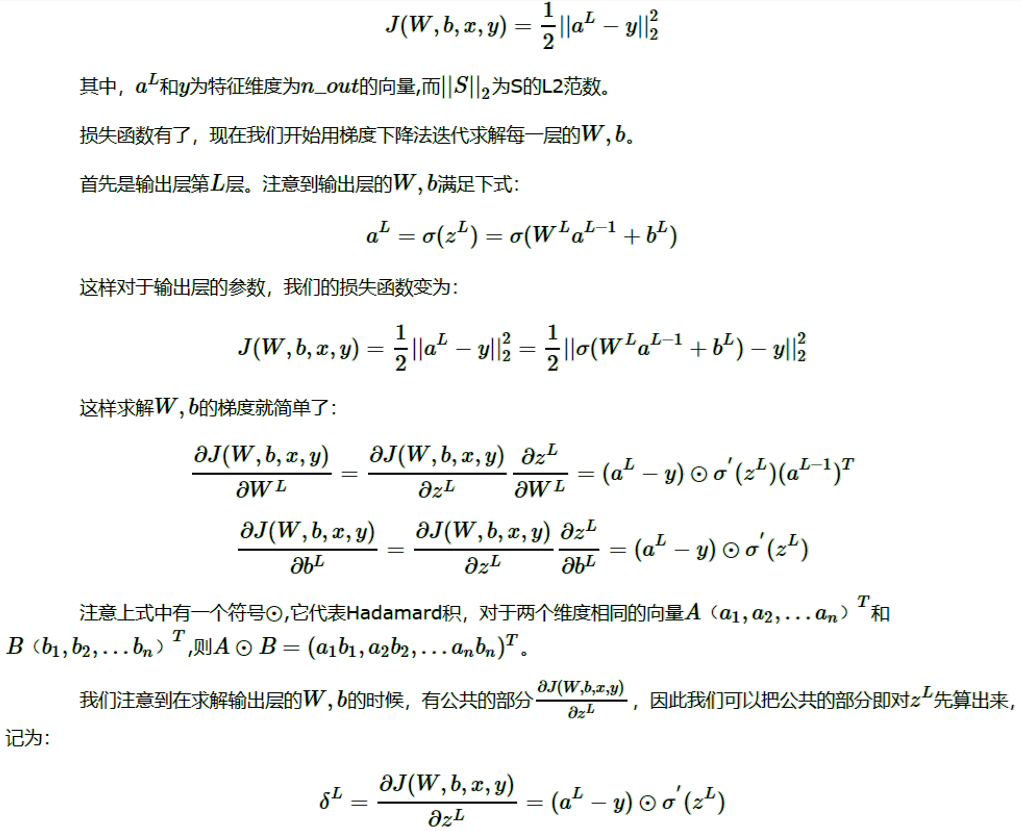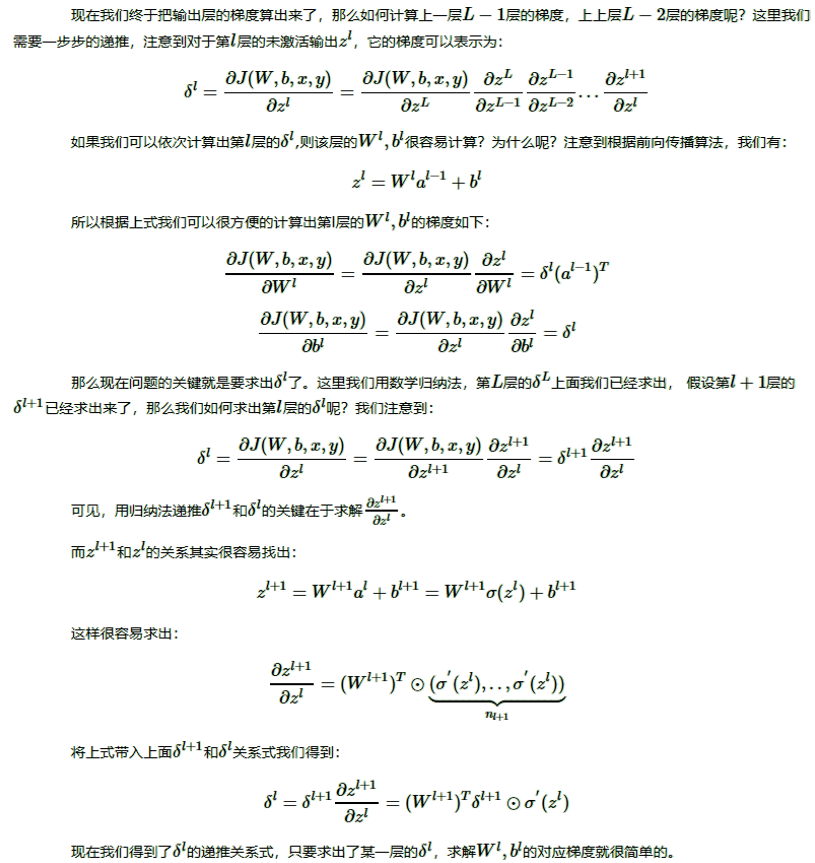2.2 DNN反向传播算法过程

梯度下降法有批量（Batch），小批量(mini-Batch)，随机三个变种，为了简化描述，这里以最基本的批量梯度下降法为例来描述反向传播算法。实际上在业界使用最多的是mini-Batch的梯度下降法。不过区别仅仅在于迭代时训练样本的选择而已。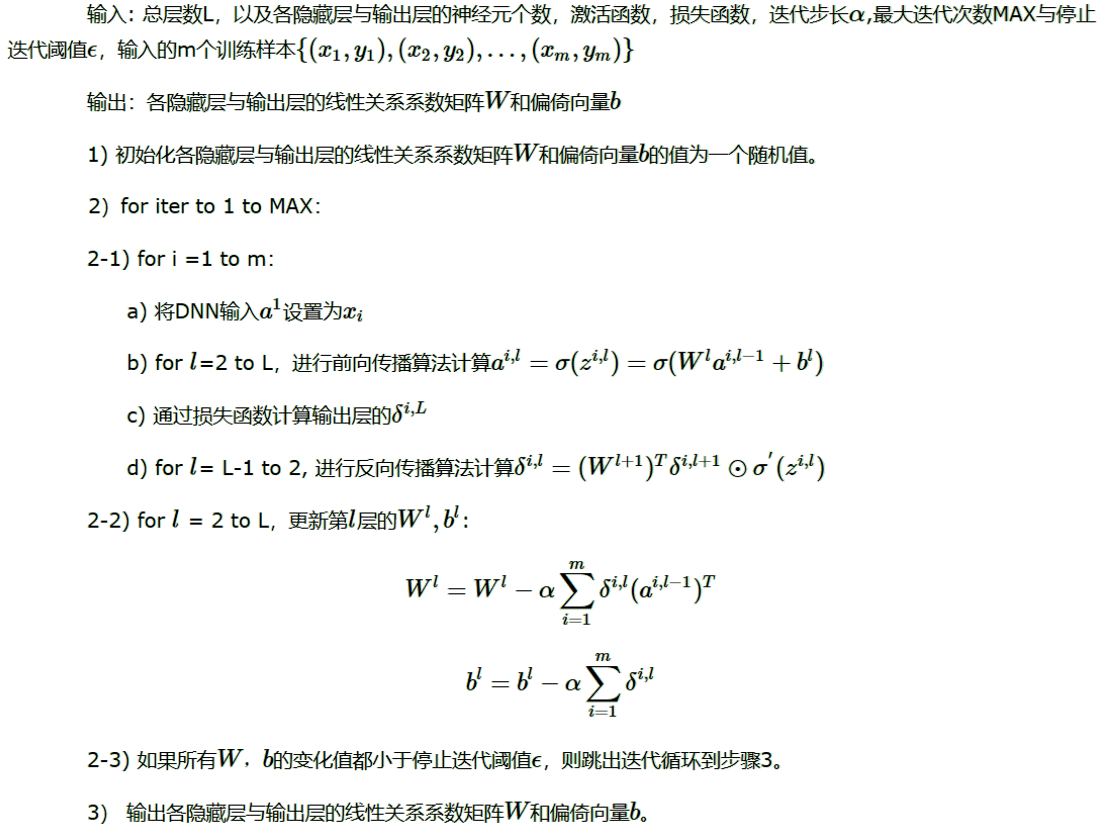3 损失函数和激活函数

3.1 均方差损失函数+Sigmoid激活函数的问题

Sigmoid激活函数的表达式和图像：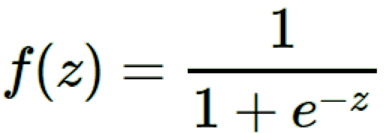对于Sigmoid，当z的取值越来越大（或z的取值越来越小时），函数曲线变得越来越平缓，导数σ′(z)也越来越小。仅仅在z取值为0附近时，导数σ′(z)的取值较大。在使用均方差+Sigmoid的反向传播算法中，每一层向前递推都要乘以σ′(z),得到梯度变化值。Sigmoid的这个曲线意味着在大多数时候，梯度变化值很小，导致W,b更新到极值的速度较慢，算法收敛速度较慢。

2. 使用交叉熵损失函数+Sigmoid激活函数改进DNN算法收敛速度

每个样本的交叉熵损失函数的形式：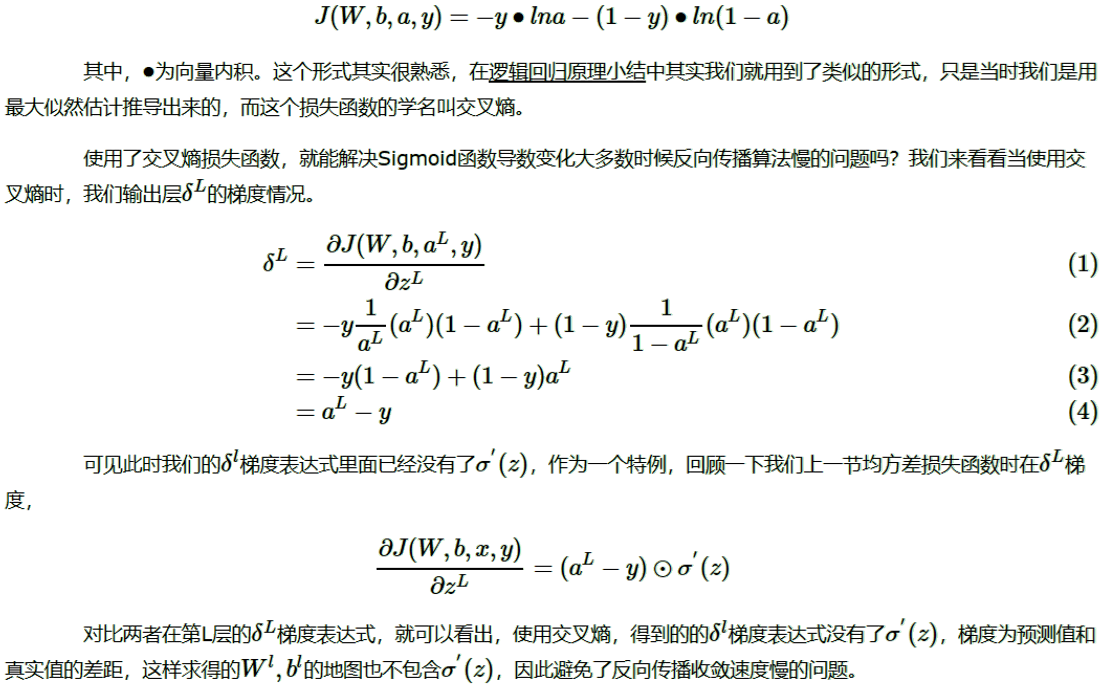另外，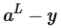表示预测值与实际值的误差，当误差越大时，梯度就越大，参数w和b的调整就越快，训练的速度也就越快。通常情况下，如果使用了sigmoid激活函数，交叉熵损失函数肯定比均方差损失函数好用。综上：如果输出神经元是线性的，那么二次代价函数就是一种合适的选择，如果输出神经元是S型函数（sigmoid，tanh），那么比较适合用交叉熵代价函数。

3. 使用对数似然损失函数和softmax激活函数进行DNN分类输出

对数似然函数与softmax的组合和交叉熵与sigmoid函数的组合相似，对数似然代价函数在二分类时可以化简为交叉熵代价函数的形式。

将DNN用于分类问题，在输出层用softmax激活函数非常常见。DNN分类模型要求是输出层神经元输出的值在0到1之间，同时所有输出值之和为1。普通DNN是无法满足这个要求。对现有的全连接DNN稍作改良，将输出层的激活函数从Sigmoid之类的函数转变为上式的softmax激活函数，即可用于解决分类问题。在现有的DNN模型中，将输出层第i个神经元的激活函数定义为如下形式：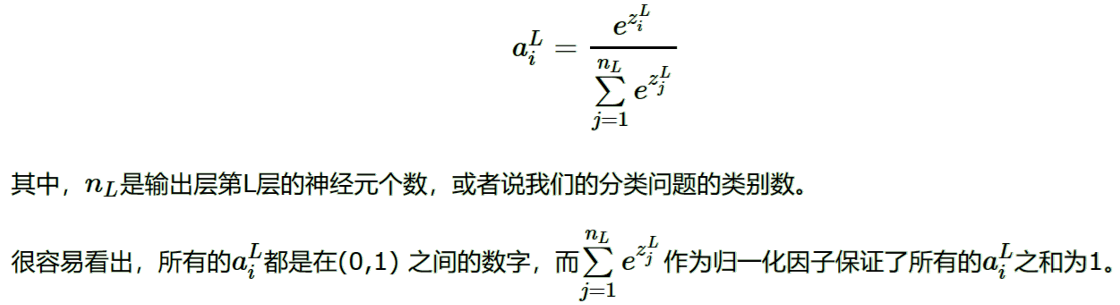softmax激活函数在前向传播算法时的使用：假设输出层为三个神经元，而未激活的输出为3,1和-3，求出各自的指数表达式为：20,2.7和0.05，归一化因子即为22.75，则三个类别的概率输出分布为0.88，0.12和0。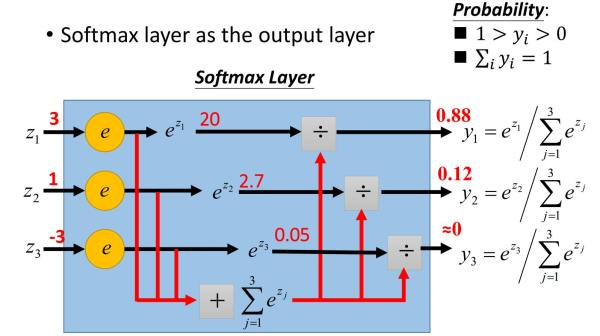4. 梯度爆炸，梯度消失与ReLU激活函数

在反向传播算法中，由于使用了是矩阵求导的链式法则，有一大串连乘，如果连乘的数字在每层都是小于1的，则梯度越往前乘越小，导致梯度消失，而如果连乘的数字在每层都是大于1的，则梯度越往前乘越大，导致梯度爆炸。对于梯度爆炸，则一般可以通过调整DNN模型中的初始化参数得以解决。

反向传播算法中δ的计算：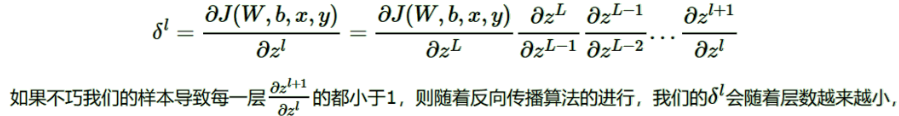甚至接近于0，导致梯度几乎消失，进而导致前面隐藏层的W，b参数随着迭代的进行几乎没有大的改变，收敛速度较慢。

一个可能部分解决梯度消失问题的办法是使用ReLU（Rectified Linear Unit）激活函数：σ(z)=max(0,z)，ReLU在卷积神经网络CNN中得到了广泛的应用。

5. DNN损失函数和激活函数小结

1）如果使用sigmoid激活函数，则交叉熵损失函数一般肯定比均方差损失函数好。2）如果是DNN用于分类，则一般在输出层使用softmax激活函数和对数似然损失函数。3）ReLU激活函数对梯度消失问题有一定程度的解决，尤其是在CNN模型中。

DNN常用的激活函数：

1）sigmoid：2）ReLU：σ(z)=max(0,z)
3） tanh：sigmoid的变种，输出区间为[-1,1]表达式为：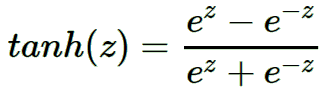tanh激活函数和sigmoid激活函数的关系为：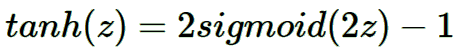4） softplus：sigmoid函数的原函数，表达式为：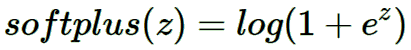它的导数就是sigmoid函数。softplus的函数图像和ReLU有些类似。它出现的比ReLU早，可以视为ReLU的鼻祖。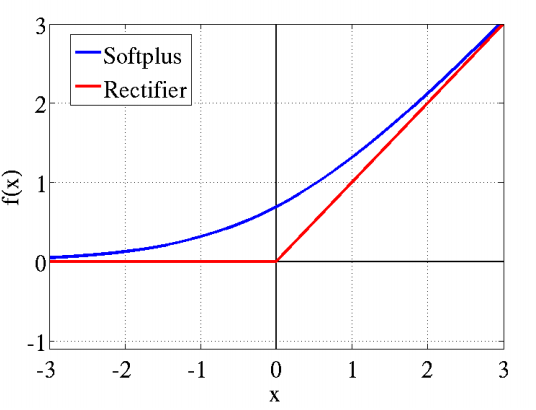5）PReLU：ReLU的变种，特点是如果未激活值小于0，不是简单粗暴的直接变为0，而是进行一定幅度的缩小。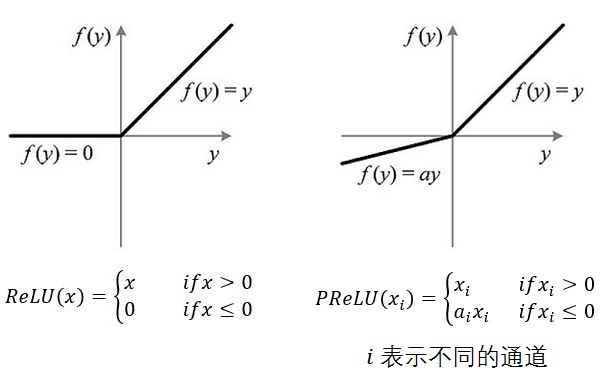正则化

1. DNN的L1&L2正则化

L1正则化和L2正则化原理类似，重点讲述DNN的L2正则化。DNN的L2正则化通常只针对与线性系数矩阵W,而不针对偏倚系数b。

假如每个样本的损失函数是均方差损失函数,则所有的m个样本的损失函数为：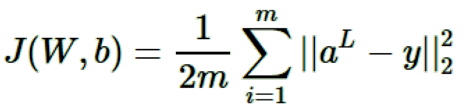则加上了L2正则化后的损失函数是：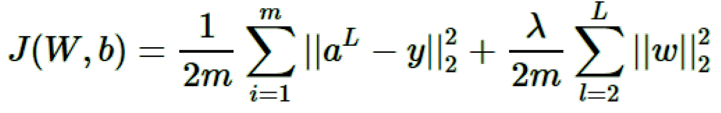其中,λ即正则化超参数，实际使用时需要调参。而w为所有权重矩阵W的所有列向量。

如果使用上式的损失函数，进行反向传播算法时，流程和没有正则化的反向传播算法完全一样，区别仅仅在于进行梯度下降法时，W的更新公式。反向传播算法中，W的梯度下降更新公式为：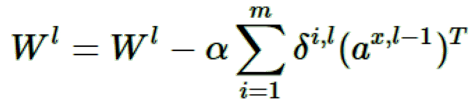加入L2正则化以后，迭代更新公式变成：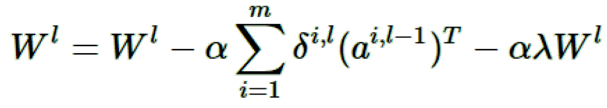注意到上式中的梯度计算中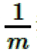我忽略了，因为α是常数，而除以m也是常数，所以等同于用了新常数α来代替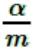。类似的L2正则化方法可以用于交叉熵损失函数或者其他的DNN损失函数。

2. DNN通过集成学习的思路正则化

除了常见的L1&L2正则化，DNN可以用Bagging的思路来正则化。常用的机器学习Bagging算法中，随机森林是最流行的。它通过随机采样构建若干个相互独立的弱决策树学习器，最后采用加权平均法或者投票法决定集成的输出。在DNN中同样可以使用Bagging的思路。不过和随机森林不同的是，这里不是若干个决策树，而是若干个DNN的网络。

首先对原始的m个训练样本进行有放回随机采样，构建N组m个样本的数据集，然后分别用这N组数据集训练DNN。即采用前向传播算法和反向传播算法得到N个DNN模型的W,b参数组合，最后对N个DNN模型的输出用加权平均法或者投票法决定最终输出。

不过用集成学习Bagging的方法有一个问题，就是DNN模型本来就比较复杂，参数很多。现在又变成了N个DNN模型，这样参数又增加了N倍，从而导致训练这样的网络要花更加多的时间和空间。因此一般N的个数不能太多，比如5-10个就可以了。

3. DNN通过dropout 正则化

Dropout指的是在用前向传播算法和反向传播算法训练DNN模型时，一批数据迭代时，随机的从全连接DNN网络中去掉一部分隐藏层的神经元。在对训练集中的一批数据进行训练时，随机去掉一部分隐藏层的神经元，并用去掉隐藏层的神经元的网络来拟合一批训练数据。如下图，去掉了一半的隐藏层神经元：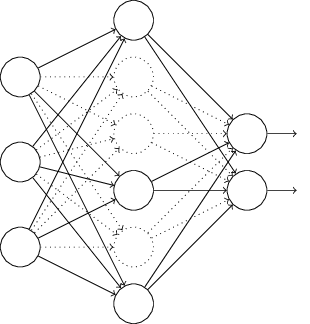然后用这个去掉隐藏层的神经元的网络来进行一轮迭代，更新所有的W,b。

dropout并不意味着这些神经元永远的消失了。在下一批数据迭代前，会把DNN模型恢复成最初的全连接模型，然后再用随机的方法去掉部分隐藏层的神经元，接着去迭代更新W,b。当然，这次用随机的方法去掉部分隐藏层后的残缺DNN网络和上次的残缺DNN网络并不相同。

总结下dropout的方法： 每轮梯度下降迭代时，它需要将训练数据分成若干批，然后分批进行迭代，每批数据迭代时，需要将原始的DNN模型随机去掉部分隐藏层的神经元，用残缺的DNN模型来迭代更新W,b。每批数据迭代更新完毕后，要将残缺的DNN模型恢复成原始的DNN模型。

dropout和Bagging的正则化思路不同，dropout模型中的W,b是共享的，所有的残缺DNN迭代时，更新的是同一组W,b；而Bagging正则化时每个DNN模型有自己独有的一套W,b参数，相互之间是独立的。相同点是：每次使用基于原始数据集得到的分批的数据集来训练模型。

使用基于dropout的正则化比基于bagging的正则化简单，当然天下没有免费的午餐，由于dropout会将原始数据分批迭代，因此原始数据集最好较大，否则模型可能会欠拟合。

4. DNN通过增强数据集正则化

增强模型泛化能力最好的办法是有更多的训练数据，但是在实际应用中，更多的训练数据往往很难得到。有时候我们不得不去自己想办法无中生有，来增加训练数据集，进而得到让模型泛化能力更强的目的。

从感知机到神经网络

感知机是二分类的线性模型，假设训练数据集是线性可分的，感知机学习的目标是求得一个能够将训练数据集正负样本点正确分开的超平面，使误分类的样本点到超平面的距离之和最小。这个模型只能用于二元分类，且无法学习比较复杂的非线性模型，因此在工业界无法使用。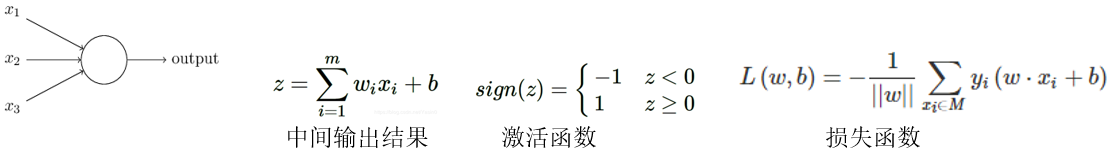将单个神经元的激活函数设为sign可以得到感知机；激活函数设为sigmoid，即可得到二分类的LR；将激活函数设为softmax可以得到多分类的LR，但需要注意的是：它们需要优化的损失函数并不相同，所以LR和感知机的不同体现在两点：激活函数和损失函数。

神经网络在感知机的模型上做了扩展，主要有三点：

加入了多层隐藏层，特征的“等级”随着网络深度的加深而变高，增强了模型的表达能力。
输出层神经元可以有多个输出，模型可以灵活的应用于分类,回归，降维和聚类等。
对激活函数做扩展。感知机的激活函数是sign(z),虽然简单但是处理能力有限，因此神经网络中一般使用：Sigmoid，Softmax，tanx, ReLU，softplus等激活函数，加入非线性因素，提高模型的表达能力。
深度神经网络（Deep Neural Networks，DNN）的基本结构

DNN可以分为：输入层，隐藏层和输出层，一般第一层是输入层，最后一层是输出层，而中间的层数都是隐藏层。层与层之间是全连接的，即第i层的任意一个神经元一定与第i+1层的任意一个神经元相连。DNN前向传播算法

从输入层开始，利用输入向量x，若干个权重系数矩阵W和偏置向量b，进行一系列线性运算和激活运算，利用上一层的输出计算下一层的输出，一层层的向后计算，一直到运算到输出层得到输出结果。

DNN反向传播算法（Back Propagation，BP）

反向传播算法的学习过程由正向传播和反向传播组成。在正向传播过程中，输入信息通过输入层，经隐藏层逐层处理并传向输出层。如果在输出层得不到期望的输出值，则取输出结果与样本标签误差的平方和作为目标函数，转入反向传播，通过对损失函数用梯度下降法进行迭代优化求极小值，找到合适的输出层和隐藏层对应的线性系数矩阵W,偏置向量b，网络的学习在权值修改过程中完成，误差达到所期望值时，网络学习结束。

DNN激活函数

为什么引入非线性激活函数

不用激活函数时，每一层输出都是上层输出的线性函数， 无论神经网络有多少层，输出都是输入的线性组合，与没有隐藏层效果相当，引入激活函数相当于加入非线性因素，可以有效避免多层网络等效于单层线性函数，提高模型表达力，使模型更有区分度。激活函数通常有如下一些性质：非线性（提高模型表达力），可微性（方便求梯度），单调性（损失函数为凸函数）

DNN常用的激活函数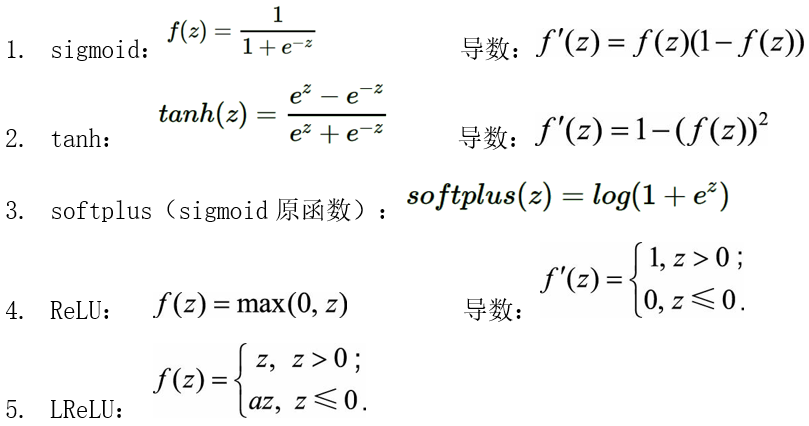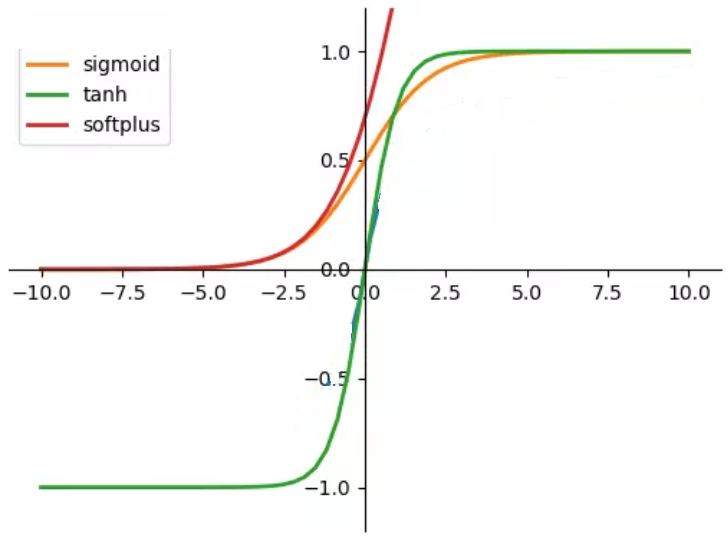梯度消失与梯度爆炸

在反向传播算法中，由于使用了矩阵求导的链式法则，后层的梯度以连乘方式叠加到前层，当神经网络中的激活函数为S型激活函数时，由于其饱和特性，在输入达到一定值的情况下，输出就不会发生明显变化，其导数逐渐趋近于0。使用梯度进行参数更新时，如果连乘的数字在每层都是小于1的，则梯度越往前乘越小，误差梯度反传到前层时几乎会衰减为0，因此无法对前层的参数进行有效的更新学习，这就会导致梯度消失，而如果连乘的数字在每层都是大于1的，则梯度越往前乘越大，导致梯度爆炸。梯度消失会导致隐层的W,b参数随着迭代的进行几乎没有大的改变，甚至不会收敛，因此无法通过加深网络层次来改善神经网络的预测效果。梯度爆炸会导致网络权重的大幅更新，引起网络不稳定，在极端情况下，权重的值变得非常大，以至于溢出，导致 NaN 值。

ReLU系列相对于Sigmoid和Tanh激活函数的优点是什么？它的缺点以及如何改进？

优点

从计算的角度上，Sigmoid和Tanh激活函数均需要计算指数，复杂度高，而ReLU只需要一个阈值即可得到激活值。
深层网络中，S型激活函数反向传播时容易出现梯度消失现象，ReLU的非饱和性可以有效地解决梯度消失的问题，提供相对宽的激活边界。
ReLU的单侧抑制会使一部分神经元的输出为 0，提供了网络的稀疏表达能力，并且减少了参数的相互依存关系，可以缓解过拟合。
缺点
使用Relu激活函数在训练过程中Relu会导致神经元不可逆死亡。因为函数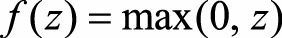会导致负梯度在经过该ReLU单元时被置为0，且在之后也不被任何数据激活，即流经该神经元的梯度永远为0，不对任何数据产生响应。在实际训练中，如果学习率（Learning Rate）设置较大，会导致超过一定比例的神经元不可逆死亡，进而参数梯度无法更新，整个训练过程失败。

缺点的改进

为解决练过程中会导致神经元死亡的问题，人们设计了ReLU的变种LReLU（ Leaky ReLU），其形式表示为：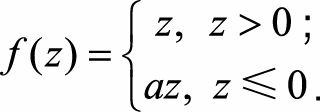LReLU与ReLU的区别在于：当z<=0时，其值不为0，而是一个斜率为a的线性函数，一般a为一个很小的正常数，这样既实现了单侧抑制，又保留了部分负梯度信息以致不完全丢失。但a值的选择增加了问题难度，需要较强的先验知识或多次重复训练以确定合适的参数值。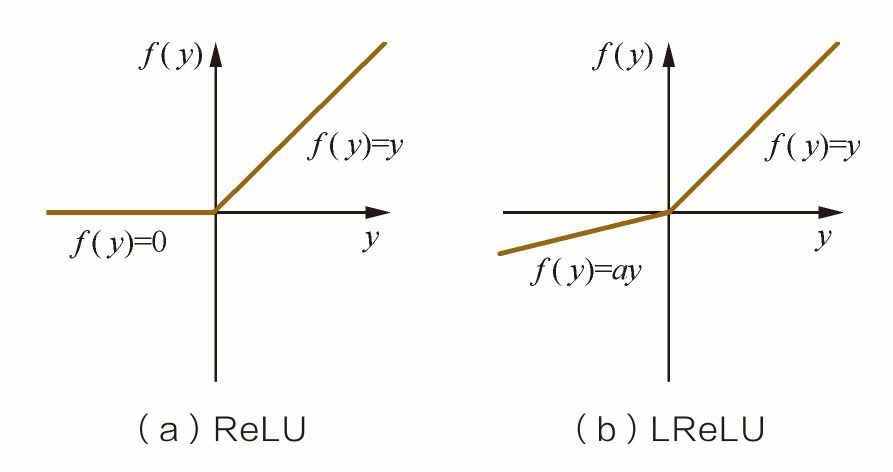DNN损失函数

常用的损失函数有：平方误差损失函数，交叉熵损失函数，对数似然损失函数

对数似然损失是对预测概率的似然估计，其最小化的本质是利用样本中的已知分布，求解导致这种分布的最佳模型参数，使这种分布出现概率最大。它衡量的是预测概率分布和真实概率分布的差异性，取值越小越好。其标准形式为：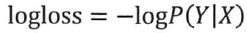。

对数似然损失函数在二分类时可以化简为交叉熵损失函数。交叉熵表示两个概率分布之间的距离，交叉熵越大，两个概率分布距离越远，概率分布越相异；交叉熵越小，两个概率分布距离越近，概率分布越相似，通过交叉熵可以判断哪个预测结果与标准答案更接近。

交叉熵损失函数的计算公式为：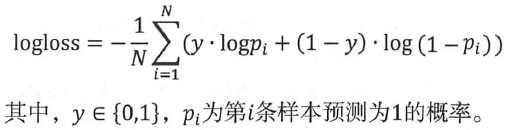对数损失在多分类问题中的计算公式为：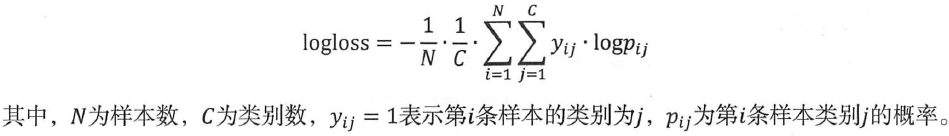平方误差损失函数和交叉熵损失函数分别适合什么场景？

一般来说，平方误差损失函数更适合输出为连续，并且最后一层不含Sigmoid或Softmax激活函数的神经网络；如果是使用Sigmoid或Softmax激活函数进行二分类或多分类的神经网络，使用交叉熵损失或对数似然损失会有更快的收敛速度。

推导平方误差损失函数相对于输出层的导数：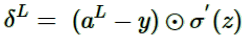其中最后一项σ′(z)为激活函数的导数。反向传播算法中，每一层向前递推都要乘以σ′(z)，得到梯度变化值，当激活函数为S型函数时，如果z 的绝对值较大，函数会趋于饱和，即σ′(z)的绝对值非常小，导致W,b更新到极值的速度较慢，算法收敛速度较慢。当使用交叉熵损失函数时，相对于输出层的导数为：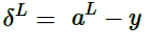（也可以认为是残差），此时的导数是线性的，因此不会存在学习速度过慢的问题，而且其表示预测值与实际值的误差，误差越大时，梯度越大，参数w和b的调整就越快，训练的速度也就越快。

将输出层的激活函数从Sigmoid之类的函数转变为Softmax激活函数可以使输出层神经元输出的值在0到1之间，同时所有输出值之和为1，可用于解决多分类问题。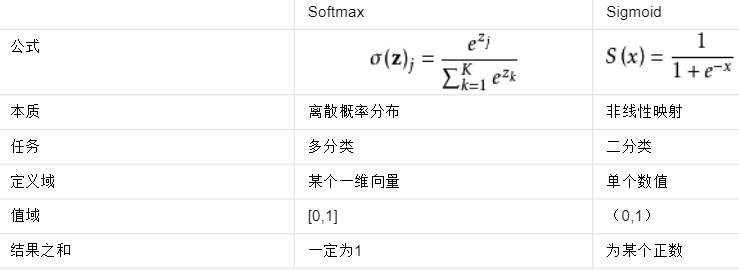DNN激活函数和损失函数小结：

如果神经元的输出是线性的，平方损失函数是一种合适的选择，如果输出神经元是S型激活函数，则交叉熵损失函数会有更快的收敛速度。
softmax激活函数与对数似然损失的组合和sigmoid函数与交叉熵的组合相似，所以一般使用sigmoid激活函数与交叉熵进行二分类输出；使用softmax激活函数与对数似然损失进行DNN多分类输出。
正则化（L1&L2，Bagging，Dropout，数据扩充）

1. DNN的L1&L2正则化

假如每个样本的损失函数是均方差损失函数,则加上了L2正则化后的损失函数是（L1正则化类似）：其中,λ即正则化超参数，实际使用时需要调参。

2. DNN通过Bagging正则化

首先对原始的m个训练样本进行有放回随机采样，构建N组m个样本的数据集，然后分别用这N组数据集训练DNN。即采用前向传播算法和反向传播算法得到N个DNN模型的W,b参数组合，最后对N个DNN模型的输出用加权平均法或者投票法决定最终输出。

需要注意的是：DNN模型本来就比较复杂，参数很多。N个DNN模型集成，参数增加了N倍，导致训练网络要花更加多的时间和空间。N的取值一般为：5-10个。

3. DNN通过Dropout 正则化Dropout（随机失活）是指在深度网络的训练中，将训练数据分成若干批，使用一批数据进行梯度下降迭代时，以一定的概率随机地 “临时丢弃”一部分神经元节点，然后用这个去掉隐藏层神经元的网络来拟合一批训练数据，并更新所有的权重和偏置（W,b）。在下一批数据迭代前，会把DNN模型恢复成最初的全连接模型，然后再用随机去掉部分隐藏层的神经元，迭代更新权重和偏置。

由于其随机丢弃部分神经元的机制，每次dropout都相当于训练了原始网络的子网络，它们共享部分权值，并且具有相同的网络层数，而模型整体的参数数目不变，这就大大简化了运算，而且这个过程会减弱神经元之间的依赖关系，减少过拟合，增强模型的泛化能力。（避免神经元相互连接，参数过多，将结果记住）

Bagging集成算法是多个模型的同时训练与测试评估，当网络与参数规模庞大时，需要消耗大量的运算时间与空间。Dropout在小批量数据集上进行操作，最后的结果相当于很多子网络的组合，这可以看做是bagging集成的近似，如果每次训练的子网络之间有一定的独立性，最后融合会降低模型的方差，增强模型的泛化能力。

Dropout和Bagging的正则化的异同

不同点：dropout模型中的W,b是一套共享的，所有的残缺DNN迭代时，更新的是同一组W,b；而Bagging正则化时每个DNN模型有自己独有的一套W,b参数，相互之间是独立的。
相同点：二者都是使用基于原始数据集分批得到的数据集来训练模型。
Dropout和 L1，L2的正则化的异同

二者的目的都是用来减少 overfitting（过拟合）。但 L1，L2正则化是针对损失函数进行优化，Dropout是改变神经网络本身的结构。

4. DNN通过扩充数据集正则化

减少模型过拟合，增强模型泛化能力最好的办法是有更多的训练数据。如：计算机视觉中可以使用图像数据增强技术。

深度学习输入数据归一化的原因（使数据处于同一数量级，具有相同的数据分布）

神经网络学习过程本质就是为了学习数据分布，一旦训练数据与测试数据的分布不同，那么网络的泛化能力也大大降低；
数据归一化可以在反向传播的过程中，加快网络中每一层权重参数的收敛速度。如果每批训练数据的分布各不相同，那么网络就要在每次迭代都去学习适应不同的数据分布，这样将会大大降低网络的训练速度
什么样的数据集不适合用深度学习？

数据集太小，深度学习容易产生过拟合。
数据集没有局部相关特性，目前深度学习表现比较好的领域主要是图像／语音／自然语言处理等领域，这些领域的一个共性是局部相关性。图像中像素组成物体，语音信号中音位组合成单词，文本数据中单词组合成句子，这些特征元素的组合一旦被打乱，表示的含义同时也被改变

展开全文• 本文重点是较为全面、系统的对深度神经网络进行通俗解释，包括网络结构、通用近似定理，神经网络模型变宽与变深及加深的问题，深度神经网络模型训练解决方案，如何让你的深度神经网络跑得更快等内容。
文章目录0 前言1 深度神经网络通俗解释2 通用近似定理—万能神经网络3 神经网络模型变宽与变深4 神经网络加深的问题5 深度神经网络模型训练解决方案5.1 训练数据集5.2 选择恰当的激励函数（activation function）5.3 隐藏单元和隐层（Hidden Units and Layers）的数量5.4 权重初始化 （Weight Initialization）5.4.1 全部初始化为零5.4.2 初始化为较小的随机数5.4.3 Xavier/Glorot Initialization5.4.4 MSRA/He initialization5.4.5 Batch Normalization5.5 学习率5.6 超参数调参：扔掉网格搜索，拥抱随机搜索5.7 学习方法5.8 无监督预训练（Unsupervised Pretraining ）5.9 Mini-Batch（小批量） 对比随机学习（Stochastic Learning）5.10 打乱训练样本5.11 使用 Dropout 正则化5.12 周期 / 训练迭代次数5.13 训练过程可视化6 如何让你的深度神经网络跑得更快6.1 参数修剪和共享6.2 低秩分解6.3 模型蒸馏
0 前言
人们对神经网络的研究已经超过半个世纪。最初时，人们研究的神经网络通常只有几层的网络，随着研究的深入，特别是深度学习的兴起，深度网络通常有更多的层数，今天的神经网络一般在五层以上，甚至多达一千多层。我们用的神经网络，通常是中间有一个隐层或者有两个隐层。今天，我们所说的深度神经网络指的是什么？直白的说就是用的神经网络隐藏层有很多层，例如，2012年ImageNet 竞赛的冠军模型AlexNet用了8层，2014年冠军模型GooleNet用了22层，2015年冠军模型RestNet用了152 层，2016年多达1207层。
我们在与学习研究神经网络对比，增加了深度求解遇到三个主问题：
一是神经网络的原生问题，求解过程中会遇到梯度消失或者梯度爆炸问题；
二是神经网络性能，训练过程太慢，耗费资源；
三是神经网络过拟合问题。
2006年，深度学习之父 Geoffrey Hinton等人利用预训练方法缓解了局部最优解问题，基于深信度网（DBN）提出非监督贪心逐层训练算法，解决深层结构训练等优化难题。将隐含层推动到了7层，至此，神经网络真正意义上有了“深度”，不过，仍没有给出“深度”等明确定义，例如在语音识别中4层网络就被认为是“较深的”，而对于图像识别模型，20多层也比较常见。为了克服梯度消失问题，用ReLU等激活函数代替了 sigmoid，形成了深度神经网络（ DNN）的基本形式。单从结构上来说，全连接的DNN和多层感知机是没有任何区别的。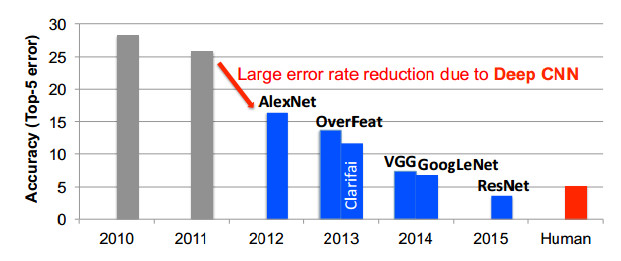我们以ImageNet竞赛为例，从各年最佳参赛者的表现来看。可以看出算法的准确情况由最初25%以上的错误率25％，到2015年，ImageNet竞赛冠军模型ResNet已经超过人类水平的错误率（5％），将错误率降到3％以下。
1 深度神经网络通俗解释
虽然根据不同应用场景，深度神经网络的结构和深度各异，但基本符合神经网络的输入层、隐藏层、输出层结构，而且，目前重点是快速演化以提升模型准确性和效率。所有深度神经网络的输入值，是一套与神经网络表征能力密切相关的信息值。这些值可以是一张图片的像素，也可以是一段音频样本的频率和振幅的数字化表示。
神经网络的层与层之间一般是全连接（FC，也指多层感知器），即第n层的任意一个神经元一定与第n+1层的任意一个神经元相连。虽然DNN从整体上看起来很庞大、复杂，但是从微观的局部模型来说，与感知机是一样，即一个线性关系加上一个激活函数。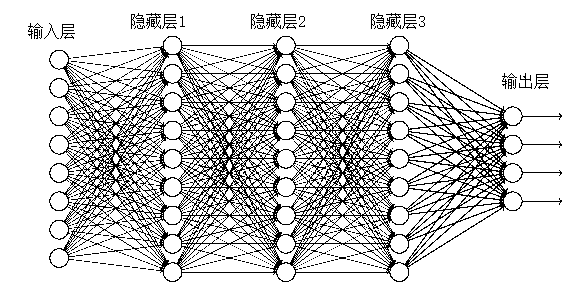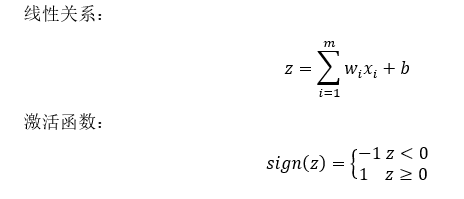输入诺干个x 经过线运算得z，激活函数的运算得到一个新的值，这个值相当于开始输入的x，用a代指。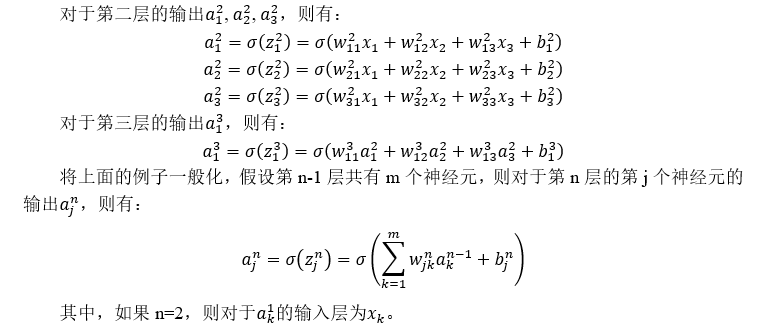我们谈论的深度学习实际指的是基于深度神经网络（deep neural networks，DNN）的学习，也就是深度人工神经网络所进行的学习过程，或称作Deep Learning。这里Deep所指的神经网络的深度就是指神经网络层数多。不过，AI业界没有清晰地、具体地给出多少层的深度网络算是深层神经网络。所以在这里我们也就不强调多深算深的概念了，我们就权且管超过2隐藏层的深度都叫深度神经网络。
实际上，DNN这个深度神经网络概念广义上一般来说CNN、RNN、GAN等都属于其范畴之内。DNN与CNN（卷积神经网络）的区别是DNN特指全连接的神经元结构，并不包含卷积单元或是时间上的关联。DNN是指包含多个隐层的神经网络，根据神经元的特点，可以分为MLP、CNNs、RNNs等，从神经元的角度来讲解，MLP是最朴素的DNN，CNNs是encode了空间相关性的DNN，RNNs是encode进了时间相关性的DNN。
在DNN中，用于处理输入的神经网络一般有两种主要结构：前馈神经网络以及循环神经网络。在前馈神经网络中，所有输入计算处理都是在前一层输出基础上进行的，直到最后一层就是深度神经网络的输出；在循环神经网络（例如LSTM ）中，网络中间环节是有内在记忆的，数据输入计算处理顺序依存上一次输出为相关影响，循环反馈作用于神经网络后输出，在这样深度神经网络中，一些中间值会被存储于网络中，也被用作与后续输入影响神经计算。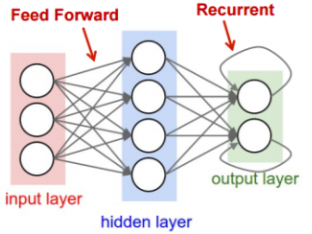用于网络链接的深度神经网络，一般可以使用全连接（FC，也指多层感知器）的形式，如图左两层间部分所示。在一个全连接层中，所有上一层的输出与下一层的所有输入都是相连接的。这样很耗费资源，占用大量的存储和计算空间。不过，在许多实际应用中，我们可以采用移除激活之间的一些连接方式，也就是将参数权重设置为0，形成一个稀疏连接层，而且这类结构化的稀疏性也不影响准确性，如果这套权重被用于每一个输入计算，就会进一步提高模型效率，显著降低权重的存储要求。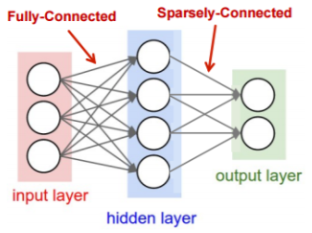2 通用近似定理—万能神经网络
通用近似定理（Universal approximation theorem，也译万能逼近定理）是指：如果一个前馈神经网络具有线性输出层和至少一层隐藏层，只要给予网络足够数量的神经元，便可以实现以足够高精度来逼近任意一个在 ℝn 的紧子集 (Compact subset) 上的连续函数。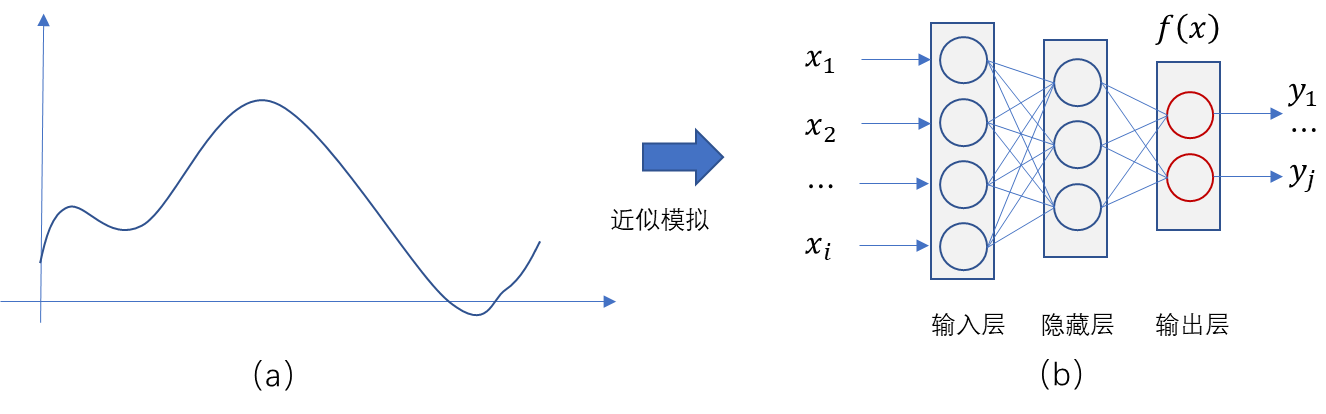如图11-5中所示神经网络通用近似模拟函数，（a）为任意函数，（b）为任意函数被神经网络以任意精度模拟。
即使函数是连续的，有关神经网络能不能解决所有问题，也是很有争议的。原因很简单，我们的理想很美好，现实问题比较多。比如，深度学习的生成对抗网络（GAN）的提出者伊恩·古德费洛（IanGoodfellow)就曾说过：“仅含有一层的前馈网络的确足以有效地表示任何函数，但是，这样的网络结构可能会格外庞大，进而无法正确地学习和泛化。
人工神经网络最神奇的地方可能就在于，它可以在理论上证明：“一个包含足够多隐含层神经元的多层前馈网络，能以任意精度逼近任意预定的连续函数”。通用近似定理告诉我们，不管函数$f(x)$在形式上有多复杂，我们总能确保找到一个神经网络，对任何可能的输入，以任意高的精度近似输出$f(x)$，即使函数有多个输入和输出，即$f(x_1,x_2,x_3⋯x_n )$。
第一层：
$h_1=f(∑_{i=1}^{m}w_i x_i+b_1 )$
第二层：
$h_2=f(∑_{j=1}^{n}w_j x_j+b_2 )$
通用近似定理的结论告诉我们，可能存在能够正确地学习和泛化的某种结构的神经网络。也就是说一个前馈神经网络如果具有线性输出层和至少一层具有任何一种激活函数（例如sigmoid激活函数）的隐藏层，只要给予网络足够数量的隐藏单元，它可以以任意的精度来近似任何从一个有限维空间到另一个有限维空间的Borel 可测函数。
3 神经网络模型变宽与变深
基于通用近似定理的理想，我们以什么样的方法，如何构建复杂的神经网络来解决问题呢？我们主要从神经网络的层数、神经元的数量两个方面入手，也就是宽度和深度是深度学习模型的两个基本维度
Goodfellow等著《深度学习》中的一张图片（图11-6），表明对某个特定问题而言，隐藏层越多，精确度越高。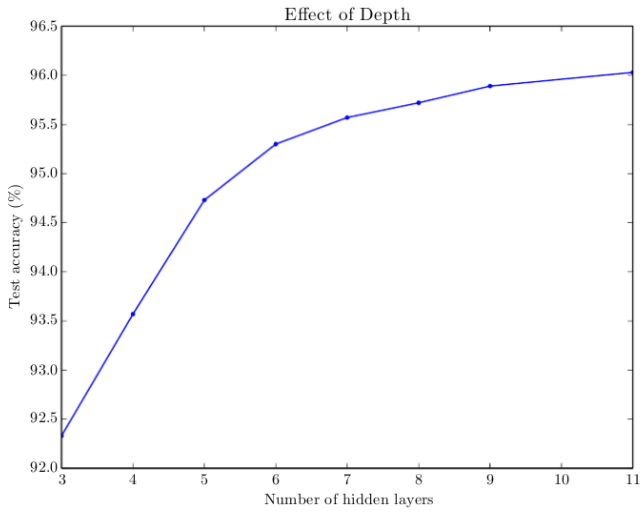我们知道理论上一个浅度神经网络可以做得和深度网络一样好，但是事实往往并非如此。为什么呢？Goodfellow等著《深度学习》为上面的问题的解答提供了一些理由。
（1）浅度网络的神经元数量将随着任务复杂度的提升进行几何级数的增长，因此浅度网络要发挥作用，会变得很大，很可能比深度网络更大。这个理由的依据是很多论文都证明了在某些案例中，浅度网络的神经元数量将随着任务复杂度的提升进行几何级数的增长，但是我们并不清楚这一结论是否适用于诸如MNIST分类和围棋这样的任务。
（2）选择深度模型编码了一个非常通用的信念，我们想要学习的函数应该涉及若干较简单的函数的组合。从表征学习的视角来说，我们相信正学习的问题包括发现一组差异的底层因素，这些因素可以进一步用其他更简单的差异的底层因素来描述。
我们要解决比较复杂的问题，要么增加深度，要么增加宽度，而增加宽度的代价往往远高于深度。
2015年，ImageNet上提出的152层的残差网络赢得了多项图像辨识竞赛的冠军。这是一个巨大的成功，看起来是一个令人难以抗拒的越深越好的论据。
在参数一样的前提下，模型深的比浅的效果更好，加大深度”就相当于函数中的模块化，也就是“并不急于解决问题，而是把问题切成比较小的问题，再解决”。而且这样的好处是：“数据集要求低，并不需要太多的数据”。
4 神经网络加深的问题
浅层神经网络可以表示的特征抽象程度不高，而层次越深，特征的抽象程度越高，也就是在某些特定任务上所谓的“效果越好”，这也是为什么深度神经网络可以做出很多只有人类才能做到的需要高度抽象理解能力的事情。
随着深度的增加，逼近函数的效果好，已经有论文证明神经网络训练的过程就是调整参数的过程，可以调整的参数（weights and bias）越多，意味着调整的自由度越大，从而逼近效果越好。
然而，预测效果却不一定好。针对同一个问题，层数少的时候效果差，这时候逐渐增加层数可以提高效果，但是如果盲目不停地增加层数，则会容易引起过拟合，从而导致预测效果不好，所以并不是层数越多，预测效果就一定会越好的。此外，添加更多层会导致更高的训练误差。
因此，神经网络随着深度的增加，实际的效果是先变好，然后再变差。例如在CIFAR-10项目上使用56层的网络其错误率，不仅在训练集上，而且在测试集上，都高于20层网络。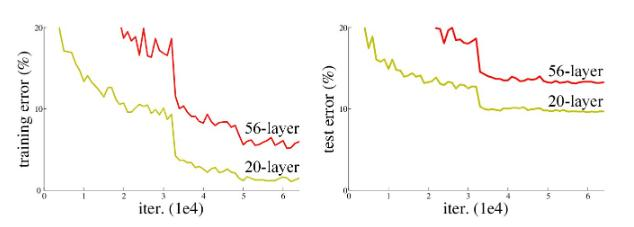这种现象的本质问题是由于出现了信息丢失而产生的过拟合问题。随着神经网络层数的加深，我们将面临三个重大问题：
（1）非凸优化问题，即优化函数越来越容易陷入局部最优解；
神经网络核心神经元是线性回归，在线性回归当中，从任意一个点出发搜索，最终必然是下降到全局最小值附近的。而在多层神经网络中，从不同点出发，可能最终困在局部最小值。局部最小值是神经网络结构带来的挥之不去的阴影，随着隐层层数的增加，非凸的目标函数越来越复杂，局部最小值点成倍增长，利用有限数据训练的深层网络，性能还不如较浅层网络。所以，从本质上来看，深度结构带来的非凸优化仍然不能解决（包括现在的各类深度学习算法和其他非凸优化问题都是如此），这限制着深度结构的发展。
随着网络层数增加，“梯度消失”（或者说是梯度发散diverge）现象更加严重。比如我们常常在多层神经网络中使用sigmoid作为神经元的激活函数。对于幅度为1的信号，在BP反向传播梯度时，每传递一层，梯度衰减为原来的1/4。这样随着层数增多，梯度指数衰减后，后面的隐层基本上接受不到有效的训练信号。那么在深度学习中是如何解决局部极值及梯度消失问题的呢？
这个问题实际上是由神经元中使用激活函数所引起的，比如多层使用Sigmoid系函数，会使得输入信号从输出层开始呈指数衰减。

在数学上，激活函数的作用就是将输入数据映射到0到1上（其中，tanh是映射-1到+1上）。至于映射的原因，除了对数据进行正则化外，大概是控制数据，使其只在一定的范围内。当然也有另外细节作用，例如Sigmoid（tanh）中，能在激活的时候，更关注数据在零（或中心点）前后的细小变化，而忽略数据在极端时的变化，例如ReLU还有避免梯度消失的作用。通常，Sigmoid（tanh）多用于全连接层，而ReLU多用于卷积层。

对于梯度消失问题， Hinton为了解决深层神经网络的训练问题，于2006年提出逐层预训练方法，比如深度信念网的训练；以及最近提出CNN中的ReLu激活函数，则从根本上提出了度消失问题的解决方案；对于深受度消失问题困扰的RNN，其变种LSTM也克服了这个问题。
（3）过拟合问题。
真实的应用中想要的并不是让模型尽量模拟训练数据的行为，而是希望通过训练出来的模型对未知的数据给予判断。模型在训练数据上的表现并不一定代表了它在未知数据上的表现。模型过拟合是神经网络庞大复杂结构和参数，以及数据集所带来的，这样结构和参数、数据集尽管使训练错误率降的很低，但是测试错误率却高的离谱，模型达不到泛化要求。
对于深度神经网络模型，与其他机器学习模型相比，一般不易过拟合。而神经网络过拟合问题，往往是在训练数据不够多，网络结构很复杂，或者过度训练时，可能会产生过拟合问题。
那么过拟合的通俗解释就是，随着对于给定的模型的训练过程的进行，在训练集上的错误率渐渐减小，但是在测试集上的错误率却反而渐渐增大。这是因为训练出来的网络过拟合了训练集，对测试集外的数据效果不好。也就是如过产生了过拟合问题，那么用训练集得到的准确率同测试集得到的准确率相差非常大。
那么为了防止过拟合问题，我们可用的方法有：增大数据集（例如增加噪声）、采用正则化方法、在网络层采用dropout方法，以及损失函数是用于优化训练数据。
5 深度神经网络模型训练解决方案
为了解决神经网络变深、结构变复杂所带来难以训练的问题，我们总结出一系列训练解决方案。
5.1 训练数据集
我们开发人员往往习惯把原始数据直接扔给DNN，在这种情况下，DNN仍然能够给出不错的结果。但是，有句老话说“给定恰当的数据类型，一个简单的模型能比复杂 DNN 提供更好、更快的结果”。我们不讨论特例，在今天，这句话仍然没有过时。因此，不管你是在计算机视觉（ CV），自然语言处理（NLP）还是统计建模（Statistical Modelling）等领域，想要对原始数据预处理，有几个方法可以得到更好的训练数据：

获取更多的数据，增大数据集；
剔除所有包含损坏数据的训练样本，比如短文字，高度扭曲的图像，假输出标签，包含许多虚值（null values）的属性；
通过数据扩张（Data Augmentation）增加样本，以图像为例，包括增加噪声、旋转图像、拉伸图像等等。

5.2 选择恰当的激励函数（activation function）
激励函数是把非线性（non-linearity）加入了神经网络模型中，是神经网络的重要核心部分。
激励函数包括Sigmoid 函数、Tanh函数、ReLu函数等等激励函数。比如多年来，很多人一直倾向的选择Sigmoid 函数，但是，Sigmoid 函数将进一步导致梯度消失，近年来，我们更多是使用ReLu函数。实际工作中，需要根据具体情况，选择适合的激励函数。
5.3 隐藏单元和隐层（Hidden Units and Layers）的数量
我们通常采用保留超出最优数量的隐藏单元方案，尽量避免出现模型欠拟合（underfitting）几率，而且任何正则化方法（ regularization method）都会处理超出的单元。
另外，当采用无监督预训练的表示时（unsupervised pre-trained representations），隐藏单元的最优数目一般会变得更大。因此，通过增加隐藏单元的数目，模型会得到所需的灵活性，以在预训练表示中过滤出最合适的信息。
对于隐层的数量，我们采用直接选择最优数目方案。正如 Yoshua Bengio 在 Quora中提到的：“你只需不停增加层，直到测试误差不再减少。”
5.4 权重初始化 （Weight Initialization）
我们常见几种权重初始化方法：全部初始化为零、初始化为随机数、Xavier/Glorot Initialization初始化、MSRA/He initialization初始化等等，常用的神经网络权重初始化方法有Xavier和MSRA。
5.4.1 全部初始化为零
这种方式最简单，我们在线性回归、logistics回归的时候，经常把参数初始化为0。
W = np.zeros(input_layer_neurons, hidden_layer_neurons)
如果是将权重W全部初始化为零，这样它们的梯度一样，那么每一层所学到的参数都是一样的，在反向传播的过程中，每一层的神经元也是相同的。因此会导致代价函数在开始的时候明显下降，但是一段时间以后，停止继续下降。
5.4.2 初始化为较小的随机数
用小的随机数字初始化权重，以打破不同单元间的对称性，权重参数随机初始化为服从均值为零和方差为1的高斯分布函数。
W = np.random.randn(input_layer_neurons, hidden_layer_neurons)
开始模型可以很好的运行一段时间，但是随着时间增加，前向传递时，方差开始减少，梯度也开始向零靠近，会导致梯度消失。特别地，当激活函数为sigmoid时，梯度接近0.5；当激活函数为时tanh，梯度接近0。
5.4.3 Xavier/Glorot Initialization
Xavier initializatio是Glorot等人为了解决随机初始化的问题提出来的另一种初始化方法，他们的思想很简单，就是尽可能的让输入和输出服从相同的分布，这样就能够避免后面层的激活函数的输出值趋向于0，适用于激活函数是sigmoid和tanh。
W = np.random.randn(input_layer_neurons, hidden_layer_neurons)* sqrt(1/input_layer_neurons)
5.4.4 MSRA/He initialization
He初始化方法适用于激活函数Relu，在初始化使得正向传播时，状态值的方差保持不变；反向传播时，关于激活值的梯度的方差保持不变。
其初始化方法为：W~N(0,√(2/n))，其中，n为第 l 层神经元个数。
W = np.random.randn(input_layer_neurons,hidden_layer_neurons)* sqrt(2/input_layer_neurons)
5.4.5 Batch Normalization
随着网络层数的增加，分布逐渐发生偏移，之所以收敛慢，是因为整体分布往非线性函数取值区间的上下限靠近。这会导致反向传播时梯度消失。BN就是通过规范化的手段，把每层神经网络任意神经元这个输入值的分布强行拉回到均值0方差1的标准正态分布，使得激活输入值落入非线性函数中比较敏感的区域。可以让梯度变大，学习收敛速度快，能大大加快收敛速度。
由于现在batch norm比较流行所以可能对初始化要求不是那么高，一般用relu的话使用he初始化会好一些，或者直接用norm batch。
使用 BN 时，减少了网络对参数初始值尺度的依赖，此时使用较小的标准差（例如0.01）进行初始化即可，初始化方法没有那么重要了。
5.5 学习率
这是训练模型最重要的超参数之一，用于调节着学习过程。如果学习率设置得太小，则模型很可能需要很长时间来收敛，如果设置得过大，损失可能将会极高。通常出事设置为0.01 的学习率比较保险。但是这不是一个严格的标准，最优学习率与实际训练模型相关。
5.6 超参数调参：扔掉网格搜索，拥抱随机搜索
网格搜索（Grid Search ）在经典机器学习中十分普遍。但它在寻找 DNN 的最优超参数方面效率较低。这主要是因为 DNN 尝试不同超参数组合所耗费的时间。随着超参数不断增长，网格搜索需要的计算性能会指数级增长。超参数组合通常在期望范围之内、从均匀分布中被选择出来。加入之前获得的知识来进一步缩小搜寻空间，也是有可能的（比如，学习率不应该太大也不应该太小）。大家发现，随机搜索比网格搜索高效地多。
有两种解决办法：
（1）如果我们的团队有之前的经验，我们可以人工对部分常见超参数调参，比如学习率、隐层数目；
（2）采用随机搜索（random search），或者随机采样代替网格搜索，来选择最优超参数。
5.7 学习方法
5.8 无监督预训练（Unsupervised Pretraining ）
不管你进行的是 NLP（自然语言处理）、计算机视觉还是语音识别等任务，无监督预训练永远能帮助你训练监督、或其他无监督模型：NLP 中词向量就（Word Vectors）无所不在；你可以用 ImageNet 的数据库，使用无监督方式对你的模型预训练，或是对于两个类别的监督分类；或是更大频域的音频样本，来在扬声器消崎模型（speaker disambiguation model）中使用该信息。
5.9 Mini-Batch（小批量） 对比随机学习（Stochastic Learning）
Mini-Batch（小批量）相对于Batch梯度下降法，是对整个训练集的一次遍历（称为一个 epoch）能做 mini-batch 个数个梯度下降。并可以一直遍历训练集，直到最后收敛到一个合适的精度。
那么如何选择mini-batch 大小？
首先，如果训练集较小，小于2000个样本，直接使用batch梯度下降法，样本集较小就没必要使用mini-batch梯度下降法。
对于样本数量较多的情况，实践经验上，一般的mini-batch大小设置为32到256，用于CPU训练环境，32到1024用于GPU训练环境，考虑到计算机内存设置和使用的方式，如果mini-batch大小是2^n，训练会运行地快一些，获得 mini-batch 的方法如下：
m = X.shape
permutation = list(np.random.permutation(m))
shuffled_X = X[:, permutation]
shuffled_Y = Y[:, permutation].reshape((1,m))

训练神经网络模型的主要目的是学习合适的参数，即训练生输入到输出的最优映射。这些参数依据每个训练样本进行调参，不管你决定使用 batch、mini-batch 还是随机学习。
mini-batch 的大小为 1，即是随机梯度下降法（stochastic gradient descent），每个样本都是独立的 mini-batch；
mini-batch 的大小为 m（数据集大小），即是 batch 梯度下降法。
5.10 打乱训练样本
这来自于信息理论（Information Theory）——“学习到一件不太可能发生的事却发生了，比学习一件很可能发生的事已经发生，包含更多的信息。”同样的，把训练样例的顺序随机化（在不同周期，或者 mini-batch），会导致更快的收敛。如果模型看到的很多样例不在同一种顺序下，运算速度会有小幅提升。
5.11 使用 Dropout 正则化
使用Dropout，就是每次随机选择一些神经元不参与训练，只有在预测的时候这些神经元才生效。
如果有数百万的参数需要学习，正则化就是避免 DNN 过拟合的必须手段。你也可以继续使用 L1/L2 正则化，但 Dropout 是检查 DNN 过拟合的更好方式。执行 Dropout 很容易，并且通常能带来更快地学习。通常Dropout 的默认值是0.5，如果模型不太复杂，Dropout 值设置0.2或许就可以。
注意，在测试阶段，Dropout 应该被关闭，权重要调整到相应大小。
5.12 周期 / 训练迭代次数
“对深度学习模型进行多个周期的训练，会得到更好的模型”——我们经常听到这句话。但多少周期才是“多”呢？其实，这里有一个简单的策略：继续按照一个固定的样例数或者周期训练模型，比如两万个样例或者一个周期。在每批样例之后，比较测试误差（test error）和训练误差（train error），如果它们的差距在缩小，那么继续训练。另外，记得在每批训练之后，保存模型的参数，所以训练好之后你可以从多个模型中做选择。
5.13 训练过程可视化
训练深度学习模型有上千种出差错的方式。我猜大家都遇到过这样的场景：模型已经训练了几个小时或者好几天，然而在训练完成之后，才意识到某个地方出问题了。为了不让你自己神经错乱，一定要对训练过程作可视化处理。比较显而易见的措施是保存或打印损失值、训练误差、测试误差等项目的日志。
6 如何让你的深度神经网络跑得更快
在我们生活的移动端，如何让深度模型在移动设备上运行，也是模型压缩加速的一大重要目标。Krizhevsky 在 2014 年的文章中，提出了两点观察结论：卷积层占据了大约 90-95% 的计算时间和参数规模，有较大的值；全连接层占据了大约 5-10% 的计算时间，95% 的参数规模，并且值较小。这为后来的研究深度模型的压缩与加速提供了统计依据。
例如有 50 个卷积层的 ResNet-50 需要超过 95MB 的存储器以及 38 亿次浮点运算。在丢弃了一些冗余的权重后，网络仍照常工作，但节省了超过 75％ 的参数和 50％ 的计算时间。
随着AI的发展，人们对AI应用需求旺盛，如何让你的深度神经网络跑得更快，这是我迫切需要的。我们的深度神经网络发展得益于计算能力的大幅提升，但是由于内存和计算能力有限，随着网络变得越来越深，而且对于移动设备在内的有严格时延要求的有限资源平台而言，神经网络压缩就成为一个关键问题。我们需要在计算能力和深度神经网络之间达到平衡，给出剪枝和共享、低秩分解、紧凑卷积滤波器、知识蒸馏等解决方案。
研究结果告诉我们，通常情况下，参数修剪和共享、低秩分解和知识蒸馏方法可以用于全连接层和卷积层的 CNN，而使用转移/紧凑型卷积核的方法仅支持卷积层。
6.1 参数修剪和共享
通俗的说，剪枝就是移除对模型性能影响不大的参数。参数的冗余和稀疏特性对模型性能的影响甚微，而剪枝正好利用了这一特性。例如在训练的很多卷积神经网络中的卷积核并非每个都是完全有用的，也就是说很多卷积核是赘余的，这有两种可能，一是去掉整个卷积核，整个都是赘余的，二是去掉卷积核中的某些权重，因为整个卷积核中的某些权重是赘余的。对于剪枝可以进一步划分为稀疏剪枝、fiters pruning、channel pruning等三类。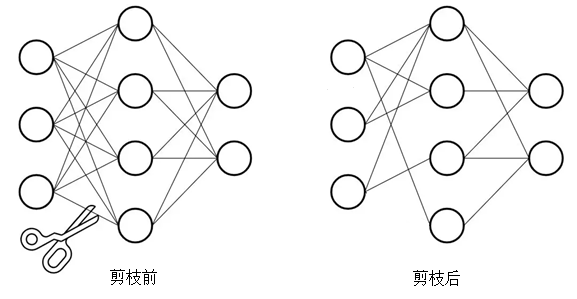（1）稀疏剪枝
在模型训练过程中，基于大小的权重剪枝会逐渐将模型权重设为零，实现模型稀疏性。稀疏模型更易于压缩，我们可以在推断过程中跳过零以缩短延迟时间。
此技术通过模型压缩改进了模型。今后，对此技术的框架支持可以缩短延迟时间。我们发现模型压缩的性能提升了6倍（Tensorflow官方网站2020年https://www.tensorflow.org/model_optimization/guide/pruning），并且对准确率造成的影响极低。
此技术正在各种语音应用（例如语音识别和文字转语音）中接受评估，并已针对各种视觉和翻译模型进行了实验。
一般情况下，可以理解为操作方式就是：

取训练好的model
取出每一个CNN的卷积核
取出每个卷积核的weight
设置一个阈值，比如接近０的阈值
每个weight进行比较比这个阈值小就直接设为０（或者接近０）即可
之后可以重新反复剪枝后retrain，之后再不断剪枝

这种方法虽然很多赘余的权重全被减掉了，对于速度，因为剪枝之后计算和存储都相当于稀疏矩阵的方式，所以有特定的加速计算的方式，对于稀疏矩阵，对于存储也是会采用０×次数类似的方式来减小尺寸，所以速度和存储大小都会被优化。
（2）channel pruning/fiters pruning
通道(channel)剪枝是压缩深度神经网络的主要方法之一。通道剪枝要在一个层上移除通道可能会急剧改变下一个层的输入。然而，通道剪枝保持了原始模型的架构，并没有引入额外的层，在GPU上的绝对加速比要更高。
一般情况下，channel pruning过程如下。

取训练好的model
取出每一个通道的
识别具有高平均百分比为零的神经元，计算通道激活为零的平均百分比
设置个阀值，例如取最高平均百分比为零通道
删除选定通道
最后生成新的model和网络文件
再训练

keras-surgeon是一个keras的模型剪枝工具，该项目支持神经元，通道以及网络层级别的剪枝操作，下面这个例子使用《Network Trimming: A Data-Driven Neuron Pruning Approach towards Efficient Deep Architectures》Hengyuan Hu（2016）等人描述的方法来确定要修剪哪些神经元。
我们定义了平均零百分比（APoZ）来度量ReLU映射后的神经元，平均零的百分比（APoZ），其中$O_{c}^{(i)}$表示第i层c通道的输出。
$APoZ_{c}^{(i)}=APoZ(O_{c}^{(i)} )=(∑_{k}^{N}∑_{j}^{M}f(O_{c,j}^{(i)} (k)=0) )/(N×M)$
其中，当为真时$f(∙)=1$，为假时$f(∙)=0$，M表示$O_{c}^{(i)}$输出特征图的维数，N表示验证示例的总数。验证实例越多，APoZ的测量越精确。
https://github.com/BenWhetton/keras-surgeon
from keras.layers import Dense, Conv2D, MaxPool2D, Flatten
from keras.models import Sequential
from keras import layers
from keras import callbacks
from tensorflow.examples.tutorials.mnist import input_data

from kerassurgeon import identify
from kerassurgeon.operations import delete_channels

def main():
training_verbosity = 2
val_images = mnist.validation.images
val_labels = mnist.validation.labels

# Create LeNet model
model = Sequential()
[3, 3],
input_shape=[28, 28, 1],
activation='relu',
name='conv_1'))

loss='categorical_crossentropy',
metrics=['accuracy'])

early_stopping = callbacks.EarlyStopping(monitor='val_loss',
min_delta=0,
patience=10,
verbose=training_verbosity,
mode='auto')
reduce_lr = callbacks.ReduceLROnPlateau(monitor='val_loss',
factor=0.1,
patience=5,
verbose=training_verbosity,
mode='auto',
epsilon=0.0001,
cooldown=0,
min_lr=0)

# Train LeNet on MNIST
results = model.fit(mnist.train.images,
mnist.train.labels,
epochs=200,
batch_size=128,
verbose=2,
validation_data=(val_images, val_labels),
callbacks=[early_stopping, reduce_lr])

loss = model.evaluate(val_images, val_labels, batch_size=128, verbose=2)
print('original model loss:', loss, '\n')

layer_name = 'dense_1'
while True:
layer = model.get_layer(name=layer_name)
apoz = identify.get_apoz(model, layer, val_images,bs=128)
high_apoz_channels = identify.high_apoz(apoz)
model = delete_channels(model, layer, high_apoz_channels)

loss='categorical_crossentropy',
metrics=['accuracy'])

loss = model.evaluate(val_images,
val_labels,
batch_size=128,
verbose=2)
print('model loss after pruning: ', loss, '\n')

results = model.fit(mnist.train.images,
mnist.train.labels,
epochs=200,
batch_size=128,
verbose=training_verbosity,
validation_data=(val_images, val_labels),
callbacks=[early_stopping, reduce_lr])

loss = model.evaluate(val_images,
val_labels,
batch_size=128,
verbose=2)
print('model loss after retraining: ', loss, '\n')
if __name__ == '__main__':
main()

安装keras-surgeon剪枝工具：
pip install kerassurgeon。
（3）过滤器剪枝（fiters pruning）
主流的滤波器剪枝（filter pruning）方法都是在第一轮训练完成之后用各种metrics（比如filter norm大小）直接剔除不那么重要的filters，并重新finetune模型以补偿剪枝过程中带来的性能损失。按soft pruning的说法，这类剪枝方法应该叫hard pruning，也就是在训练之后强行将一部分filters剔除。
一般情况下，fiters pruning过程如下。

取训练好的model
取出每一个CNN的卷积核
计算每个卷积核的所有权重之和大小
设置一个阈值，比如为１
如果这个卷积核的权重值和小于１则直接剔除掉
最后生成新的model和网络文件
再训练

这种方法可以瞬间减小你的model的尺寸，并且加快速度，因为你的计算量明显减小了，并且model中的卷积核的个数也少了，也就是说你总体的权重少了，剩下来的都是默认有用的卷积核或者权重，但是问题来了，就是精度会大大降低，因为这个也没告诉你怎么剪枝，具体每层每层之间怎么裁剪，只能不断尝试。对于channel，这边的channel就是某个卷积层通过乘以每个卷积核得到的那些feature channel，来判断这些channel哪些是冗余的方式来消除filters。
注意：前面一两层最好不剪枝，或者少量剪枝；和SSD有关联的层尽量不剪枝，或者少剪枝；剪枝之后一定要再训练，acc会上升一些，但是不要次数过多，会过拟合。
（4）量化和二进制化
量化就是将无限域的值转化为有限域离散值的过程。假设我们有一张灰度图像。量化（N级）就是将图像中的每个像素点的颜色用N份权重来表示。而二进制化只会给出图像的两个灰度级别（灰或非灰）。
网络量化通过减少表示每个权重所需的比特数来压缩原始网络。量化限制了可用于我们内核中的不同权重数目。对于N个比特位，可以表示2的N次方个权重。我们的目的是修改内核中的权重只能取2的N次方个值。因此，低于四个比特位参数量化虽然准确率没有受到很大损失，但却很难表示权重。
6.2 低秩分解
低秩分解的方法其实就是运用了矩阵分解和矩阵乘法的结合律。实际上就是把较大的卷积核分解为两个级联的行卷积核和列卷积核。常见的就是一个3×3的卷积层，替换为一个3×1的卷积层加上一个1×3的卷积核。
低秩估计的方法的优势在于，没有改变基础运算的结构，不需要额外定义新的操作。分解后的网络仍是用卷积操作来实现的，所以其适用面比较广泛。分解方法多种多样，但一般分解后的网络都需要参数调优，以保证分解后网络模型的准确率。
常见的低秩分解有：奇异值分解SVD、CP分解、Tucker分解、Tensor Train分解和Block Term分解等，用低秩矩阵近似原有权重矩阵。
6.3 模型蒸馏
理想AI模型的目标不是拟合训练数据，而是学习如何泛化到新的数据。所以蒸馏的目标是让student学习到teacher的泛化能力，理论上得到的结果会比单纯拟合训练数据的student要好。另外，对于分类任务，如果soft targets的熵比hard targets高，那显然student会学习到更多的信息。
模型蒸馏也叫知识蒸馏，是Hinton在NIPS2014提出了知识蒸馏（Knowledge Distillation）的概念，最基本的想法就是将大模型学习出来的知识作为先验，将先验知识传递到小规模的神经网络中，之后实际应用中部署小规模的神经网络。这样做有三点依据：

大规模神经网络得到的类别预测包含了数据结构间的相似性；
有了先验的小规模神经网络只需要很少的新场景数据就能够收敛；
Softmax函数随着温度变量（temperature）的升高分布更均匀。

对于网络压缩和加速，模型蒸馏则直接设计了一个简单结构的小网络，那小网络的准确率怎么和大网络比呢？Hinton前辈提出了一个非常简单且有效的方法——网络蒸馏。主要思想是用预训练好的网络，是指通常结构较复杂，准确率较高的网络，来指导小网络的训练，并使小网络达到与复杂网络相近的准确率。大网络类比于老师，小网络类比于学生，老师经过漫长时间的“训练”摸索出一套适用于某个任务的方法，于是将方法提炼成“知识”传授给学生，帮助学生更快地学会处理相似的任务。整个思想中最大的难题在于如何有效地表达“知识”，并有效地指导小网络的训练。


展开全文tensorflow
• ## 深度神经网络DNN

千次阅读 2019-02-28 01:14:11
深度网络DNN的概念，是基于浅层网路——多层感知机MLP（或称人工神经网络ANN）的基础上发展而来。关于MLP或ANN的知识，此处不作赘述，网上有很多资料可以参考。 DNN是一个很广的概念，大名鼎鼎的CNN、RNN、GAN等都...
深度网络DNN的概念，是基于浅层网路——多层感知机MLP（或称人工神经网络ANN）的基础上发展而来。关于MLP或ANN的知识，此处不作赘述，网上有很多资料可以参考。

DNN是一个很广的概念，大名鼎鼎的CNN、RNN、GAN等都属于其范畴之内。CNN多用于网络结构数据类型的任务，如图像、声音、文本等；RNN多用于时间序列类型的任务，如音频、文本（NLP）、视频等；GAN则主要用于“生成数据、以假乱真”，适用于创作类任务。但是本文亦不叙述CNN等知识，相关知识可参考本人相关博客卷积神经网络CNN。本文主要讲述一般的DNN任务，考虑如下问题：

有8000个变量，其中少数变量之间可能存在相关性；有20000个样本，每个样本都有这8000个变量的数据；这20000个样本可以被分为2类（label）。求如何用这些变量构造一个分类器？

这个问题，是一个典型的高通量信息数据的问题（如基因组学、代谢组学等），首先考虑的方法是普通机器学习的模型，如PCA、PLS、LASSO等降维，单因素差异分析如Wilcoxon检验、Fold Change卡差异界值，甚至聚类分析，再建模弄个SVM、随机森林、ANN等，最后回归解释性模型如Logistic等。机器学习进阶一点的方法还有XGBoost也可以试试。

但是，如果抛开普通机器学习的方法，仅讨论深度学习的方法，这个任务该如何解决？有两个idea，第一是直接套用CNN，但是直观感觉，卷积在此处的作用可能不大（也可能有用，将变量固定顺序，将构成一维图像，虽然相邻像素较难联合构成有效的局部特征），因此CNN的方法试试就行；第二是采用全连接的DNN（如FC的ResNet），由于是全连接，参数较多，容易过拟合，考验炼丹的能力了（注意ReLU等非线性变换需要保留，否则就退化成线性分类器了）。

深度学习就是学习如何利用矩阵的线性变换加激活函数的非线性变换，将原始输入空间投向线性可分/稀疏的空间去分类/回归。增加节点数：增加维度，即增加线性转换能力。增加层数：增加激活函数的次数，即增加非线性转换次数。

深度 vs. 浅层：浅层神经网络可以模拟任何函数，但数据量的代价是无法接受的。深层解决了这个问题。相比浅层神经网络，深层神经网络可以用更少的数据量来学到更好的拟合。深层的前提是：空间中的元素可以由迭代发展而来的。

有人总结了一下经验（看看就好，也不要全信）：

1.如果数据已经有了特征，比如性别、年龄、地点、访问量等等特征，则直接使用DNN或者全连接进行判断；

2.在有了特征的情况下使用CNN可能会导致模型的准确率不是特别高。

本文就这类问题，进行建模技巧讲解。

从Neural Network到Deep Learning

本部分摘自：DNN与ANN的区别

传统神经网络中，采用的是BP算法的方式进行，简单来讲就是采用迭代的算法来训练整个网络，随机设定初值，计算当前网络的输出，然后根据当前输出和label之间的差去改变前面各层的参数，直到收敛（整体是一个梯度下降法）。

大约二三十年前，Neural Network曾经是ML领域特别火热的一个方向，但是后来确慢慢淡出了，原因包括以下几个方面：

1）比较容易过拟合，参数比较难tune，而且需要不少trick；

2）训练速度比较慢，在层次比较少（小于等于3）的情况下效果并不比其它方法更优；

同时BP算法存在的问题：

（1）梯度越来越稀疏：从顶层越往下，误差校正信号越来越小；

（2）收敛到局部最小值：尤其是从远离最优区域开始的时候（随机值初始化会导致这种情况的发生）。

直到2006年，Hinton等人提成了一个实际可行的Deep Learning框架。

Hinton等人提出，在非监督数据上建立多层神经网络的一个有效方法，简单的说，分为两步，一是每次训练一层网络，二是调优，使原始表示x向上生成的高级表示r和该高级表示r向下生成的x'尽可能一致。方法是：

1）首先逐层构建单层神经元，这样每次都是训练一个单层网络。

2）当所有层训练完后，Hinton使用wake-sleep算法进行调优。

将除最顶层的其它层间的权重变为双向的，这样最顶层仍然是一个单层神经网络，而其它层则变为了图模型。向上的权重用于“认知”，向下的权重用于“生成”。然后使用Wake-Sleep算法调整所有的权重。让认知和生成达成一致，也就是保证生成的最顶层表示能够尽可能正确的复原底层的结点。比如顶层的一个结点表示人脸，那么所有人脸的图像应该激活这个结点，并且这个结果向下生成的图像应该能够表现为一个大概的人脸图像。Wake-Sleep算法分为醒（wake）和睡（sleep）两个部分。

1）wake阶段：认知过程，通过外界的特征和向上的权重（认知权重）产生每一层的抽象表示（结点状态），并且使用梯度下降修改层间的下行权重（生成权重）。也就是“如果现实跟我想象的不一样，改变我的权重使得我想象的东西就是这样的”。

2）sleep阶段：生成过程，通过顶层表示（醒时学得的概念）和向下权重，生成底层的状态，同时修改层间向上的权重。也就是“如果梦中的景象不是我脑中的相应概念，改变我的认知权重使得这种景象在我看来就是这个概念”。

Deep Learning训练过程具体如下：

1）使用自下上升非监督学习（就是从底层开始，一层一层的往顶层训练）：

采用无标定数据（有标定数据也可）分层训练各层参数，这一步可以看作是一个无监督训练过程，是和传统神经网络区别最大的部分（这个过程可以看作是feature learning过程）：

具体的，先用无标定数据训练第一层，训练时先学习第一层的参数（这一层可以看作是得到一个使得输出和输入差别最小的三层神经网络的隐层），由于模型capacity的限制以及稀疏性约束，使得得到的模型能够学习到数据本身的结构，从而得到比输入更具有表示能力的特征；在学习得到第n-1层后，将n-1层的输出作为第n层的输入，训练第n层，由此分别得到各层的参数；

2）自顶向下的监督学习（就是通过带标签的数据去训练，误差自顶向下传输，对网络进行微调）：

基于第一步得到的各层参数进一步fine-tune整个多层模型的参数，这一步是一个有监督训练过程；第一步类似神经网络的随机初始化初值过程，由于DL的第一步不是随机初始化，而是通过学习输入数据的结构得到的，因而这个初值更接近全局最优，从而能够取得更好的效果；所以deep learning效果好很大程度上归功于第一步的feature learning过程。

一些技巧和认知的梳理

本部分摘自：面试常问的深度学习(DNN、CNN、RNN)的相关问题

一、如何避免陷入局部极小值

1.调节步伐：调节学习速率，使每一次的更新“步伐”不同；

2.优化起点：合理初始化权重（weights initialization）、预训练网络（pre-train），使网络获得一个较好的“起始点”，如最右侧的起始点就比最左侧的起始点要好。常用方法有：高斯分布初始权重（Gaussian distribution）、均匀分布初始权重（Uniform distribution）、Glorot 初始权重、He初始权、稀疏矩阵初始权重（sparse matrix）。

二、如何防止过拟合
L2正则化，Dropout(若规律不是在所有样本中都存在,则dropout会删除这样的规律)，每个epoch之后shuffle训练数据，设置early-stopping。加Batch Normalization(BN首先是把所有的samples的统计分布标准化，降低了batch内不同样本的差异性，然后又允许batch内的各个samples有各自的统计分布)，BN最大的优点为允许网络使用较大的学习速率进行训练，加快网络的训练速度（减少epoch次数），提升效果。

三、为何使用Batch Normalization

若用多个梯度的均值来更新权重的批量梯度下降法可以用相对少的训练次数遍历完整个训练集，其次可以使更新的方向更加贴合整个训练集，避免单个噪音样本使网络更新到错误方向。然而也正是因为平均了多个样本的梯度，许多样本对神经网络的贡献就被其他样本平均掉了，相当于在每个epoch中，训练集的样本数被缩小了。batch中每个样本的差异性越大，这种弊端就越严重。一般的解决方法就是在每次训练完一个epoch后，将训练集中样本的顺序打乱再训练另一个epoch，不断反复。这样重新组成的batch中的样本梯度的平均值就会与上一个epoch的不同。而这显然增加了训练的时间。同时因为没办法保证每次更新的方向都贴合整个训练集的大方向，只能使用较小的学习速率。这意味着训练过程中，一部分steps对网络最终的更新起到了促进，一部分steps对网络最终的更新造成了干扰，这样“磕磕碰碰”无数个epoch后才能达到较为满意的结果。

为了解决这种“不效率”的训练，BN首先是把所有的samples的统计分布标准化，降低了batch内不同样本的差异性，然后又允许batch内的各个samples有各自的统计分布。

四、神经元的运算逻辑

本段摘自：【Keras】DNN神经网络模型 （文中附有Keras的代码实现）

神经元的运算逻辑，一定是线性内核和非线性激活相结合，所以神经元的算法是非线性的。因此DNN神经元的计算内核为X*W+b，以softmax函数（二元分类中使用Sigmoid函数）为非线性核的构造方式。同理，RNN的核采用RNN运算内核，CNN采用卷积运算内核。

逻辑分类可以视为一层DNN神经网络，计算内核为X*W+b，以softmax函数（二元分类中使用Sigmoid函数）为非线性核的构造方式。像逻辑分类这种，线性运算单元设计为权重相乘的，并且层与层之间的神经元全部相连的神经网络就是全连接神经网络，即DNN。

进一步增加隐层，，容纳更多的神经元，来增强模型的能力。比起浅层模型在特征工程和模型工程的各种尝试，神经网络通过更多的神经元直接增强模型的能力。

DNN vs. CNN：个人观点，阉割掉卷积操作、改为全连接，即可转变为普通DNN。而CNN的ResNet比较成熟，因此反而可以通过改造CNN来得到DNN（纯属个人观点，具体实现当然会有问题）

五、本质的探讨

为什么神经网络高效：并行的先验知识使得模型可用线性级数量的样本学习指数级数量的变体。

为什么深层神经网络比浅层神经网络更高效：迭代组成的先验知识使得样本可用于帮助训练其他共用同样底层结构的样本。

神经网络在什么问题上不具备优势：不满足并行与迭代先验的任务。（本人不太理解）

对于这些关于本质的说法以及神经网络在什么问题上不具备优势，本人暂时存疑。

六、训练DNN的一些其他技巧参考

如何正确训练DNN 、DNN训练技巧（Tips for Training DNN）等

这些博文中引用了一个很重要的观点：“Do not always blame overfitting”。具体的，之后展开叙述。

代码实现

待更新


展开全文• 分布式深度神经网络不仅能够允许在云端进行深度神经网络的推理，还允许在边缘和终端设备上使用神经网络的浅层部分进行快速、本地化推理。在可伸缩的分布式计算层次结构的支持下，DDNN可以在神经网络规模上进行扩展和...分布式
•     本文提出了一个多任务深度神经网络(MT-DNN)，用于跨多个自然语言理解(NLU)任务学习表示。MT-DNN不仅利用了大量的跨任务数据，而且还受益于正则化效应，从而产生更通用的表示，以帮助适应新的任务和领域。MT-...自然语言处理
• Geoffrey Hinton提出了一种名为“深度信念网络”的神经网络，可以...深度学习主要包括深度神经网络DNN、卷积神经网络CNN、循环神经网络RNN、LSTM以及强化学习等。 深度学习之所以能够成功，是因为解决了神经网络的训
•数学
• 深度神经网络算法分析 人工智能的分类 弱人工智能：特定任务与人类智力或者效率持平 通用人工智能：具有人类智力水平，解决通用问题 超人工智能：超过人类智力水平，可以在创造力上超过常人 机器学习的类型...
• 神经网络的调试基本上难于绝大多数的程序，因为大部分的神经网络的错误不会以类型错误或运行时错误显现，他们只是使得网络难以收敛。如果你是一个新人，这可能会让你非常沮丧。一个有经验的网络训练者可以系统的克服...调试
• 如果你使用百度查找的相关资料，则使用了百度的神经网络，该神经网络会根据给出的关键词对相关度最高的网页进行排名。如果你最近要买东西访问过淘宝官网，那么该网站推荐的所有产品都是由神经网络管理的。 ...
• 刚接触深度学习时，我们经常会看到神经网络、深度神经网络、卷积神经网络这些词。初看可能一头雾水，没关系，下面我们就来了解一下这些词背后的意义吧。 图1 神经元的结构 神经元（Neuron）： 从生物上来说...
• 这里是翻译kaldi主页的深度神经网络这部分，主要包括karel的版本和dan的版本，由于现在更新了新的版本，所以重新翻译。这些也将在kaldi的中文版本gitbook中发布，如果你想加入，你与我们取得联系，为kaldi的中文版做...
• 相比较而言， 利用多层MLP的微型网络， 对每个局部感受野的神经元进行更加复杂的运算， 而以前的卷积层， 局部感受野的运算仅仅只是一个单层的神经网络。 在MLP网络中比较常见的是使用一个三层的全连接网络结构， ...csdn 人工智能
• TensorFlow 中的深度神经网络 你已经学过了如何用 TensorFlow 构建一个逻辑分类器。现在你会学到如何用逻辑分类器来构建一个深度神经网络。 详细指导 接下来我们看看如何用 TensorFlow 来构建一个分类器来...class 机器学习 github
• 挑战深度学习 《深度森林：探索深度神经网络以外的方法》 阅读量：1306 收藏本文 深度神经网络的巨大成功掀起了一股深度学习热潮。 　或许，这股热潮有些“太热”了。 　就在这周，...
• 挑战深度学习 《深度森林：探索深度神经网络以外的方法》 阅读量：1306 收藏本文 深度神经网络的巨大成功掀起了一股深度学习热潮。 　或许，这股热潮有些“太热”了。 　就在这周，...
• 神经网络的调试基本上难于绝大多数的程序，因为大部分的神经网络的错误不会以类型错误或运行时错误显现，他们只是使得网络难以收敛。如果你是一个新人，这可能会让你非常沮丧。一个有经验的网络训练者可以系统的克服...调试 网络
• 点击上方“AI公园”，关注公众号，选择加“星标“或“置顶”作者：Lilian Weng编译：ronghuaiyang导读如果你和我一样，不明白为什么深度神经网络可以推广到...
• 本文转载自：深度神经网络全面概述：从基本概念到实际模型和硬件基础本文旨在提供一个关于实现 DNN 的有效处理（efficient processing）的目标的最新进展的全面性教程和调查。作者：机器之心编译| 深度神经网络(DNN)...神经网络 机器学习 深度学习
• sigmoid 函数 神经网络中经常使用的一个激活函数就是下式表示的sigmoid 函数 （sigmoid function）： h(x)=11+e−x h(x)=\frac{1}{1+e^{-x}} h(x)=1+e−x1​ 式中 e是纳皮尔常数2.7182 . . .神经网络中用sigmoid ...python
• 深度学习之所以能够成功，是因为解决了神经网络的训练问题，使得包含多个隐含层的神经网络模型变得可能。神经网络训练问题的解决，包括了四个方面的因素： （1）硬件设备特别是高性能GPU的进步，极大地提高了数值...正则化 dropout 数字识别
• Airfraft Type Recognition Based on Segmentation With Deep Convolutional Neural Networks 2018 IEEE ...为了解决这一问题，我们提出了一种基于深度卷积神经网络的新型飞机型号识别框架。首先，...图像分割 关键点检测
• 这里将自己做的一个PPT纪录一下，根据斯坦福大学CS234 lecture 5 整理而来Some of the content for this lecture is borrowed from Hugo Larochelle 神经网络相对于训练集：太小（欠拟合），找到的规律模型未能够很...反向传播
• 与仅包含一层隐含层的网络不同，深度神经网络一般采用的激活函数为ReLU而不是Sigmoid函数，并且没有了阈值，而在每一层的输出上加上了偏置（Bias）。对于三层神经网络中隐层的节点，其输入首先要与阈值作差，然后将...
• （十四）深度神经网络模型用于评分卡模型（理论） 本篇文章主要讲解以下两个内容 神经网络的概述 深度神经网络模型用于违约概率预测（代码实现看下一篇博客） 神经网络的概述 什么是人工神经网络 神经网络...人工神经网络优缺点 激活函数
• 一个是视觉互动网络 VIN，能够预测视觉场景中各个物体在未来几百步所处位置，另一个则是模块化的、具有关系推理能力的深度神经网络架构 RN，可以“即插即用”，提升其他深度神经网络结构（如 CNN）关系推理的能力。...ai class
• 引用本文： ... 深度神经网络压缩与加速综述[J]. 计算机研究与发展, 2018, 55(9): 1871-1888. Ji Rongrong,Lin Shaohui,Chao Fei,Wu Yongjian,Huang Feiyue. Deep Neural Network Compression ......